## Yet Another Titanic Solve

##### March 30, 2018

tl;dr: Another titanic solve. Uses `caret` and `tidyverse` for everything. Best model is elastinet and gets me a 0.72-0.76 score on kaggle. Enjoy.

# Objectives

1. End to end analysis using R
2. Learn the `caret` package for ML
3. Learn to present the case using R Notebooks

# Read in the dataset

I stored the raw files on Github, so I used RCurl with Wehrley’s method that utilizes read.csv to the fullest. It’s one of the best ways I’ve found to read in data and also set data-types at the same time. He’s done a great job on that function. The dataset contains one ID variable, one response variable and ten predictor variables.

``````library(RCurl,quietly = T)
library(tidyverse,quietly = T)
library(ggplot2,quietly = T)
library(gridExtra,quietly = T)
library(beanplot,quietly = T)
library(caret,quietly = T)
library(stringr,quietly = T)
library(party, quietly = T)
library(xgboost, quietly = T)
library(skimr, quietly = T)
library(alluvial, quietly = T)
library(pROC, quietly = T)
library(ggrepel, quietly = T)

readData <- function(path.name, file.name, column.types, missing.types) {
gurl <- paste(path.name,file.name,sep="")
na.strings=missing.types))
}

Titanic.path <- "https://raw.githubusercontent.com/rsangole/Titanic/master/"
train.data.file <- "train.csv"
test.data.file <- "test.csv"
missing.types <- c("NA", "")
train.column.types <- c('integer',   # PassengerId
'factor',    # Survived
'factor',    # Pclass
'character', # Name
'factor',    # Sex
'numeric',   # Age
'integer',   # SibSp
'integer',   # Parch
'character', # Ticket
'numeric',   # Fare
'character', # Cabin
'factor'     # Embarked
)

test.column.types <- train.column.types[-2]     # # no Survived column in test.csv
train.raw <- readData(path.name = Titanic.path,
file.name = train.data.file,
column.types = train.column.types,
missing.types = missing.types)
kaggletest.raw <- readData(path.name = Titanic.path,
file.name = test.data.file,
column.types = test.column.types,
missing.types = missing.types)

prep_data <- function(D) {
if (!is.null(D\$Survived)) {
D\$Survived <- factor(D\$Survived,
levels = c(1, 0),
labels = c('Survived', 'Dead'))
}
D\$Pclass <- factor(D\$Pclass,
levels = c(1, 2, 3),
labels = c('P1', 'P2', 'P3'))
D\$PassengerId <- NULL
D
}

train.raw <- prep_data(train.raw)
kaggletest.raw <- prep_data(kaggletest.raw)``````

# Train-Test Split

Here, I’m splitting up the `train` dataset into a training and a testing dataset. Right now, I don’t intend to upload any results back into Kaggle, so I’m just going to evaluate my models on my testing split.

``````set.seed(1071)
training_rows <- caret::createDataPartition(y = train.raw\$Survived, p = 0.7, list = F)
test.raw <- train.raw %>% filter(!(rownames(.) %in% training_rows))
train.raw <- train.raw %>% filter(rownames(.) %in% training_rows)
dim(train.raw)``````
``##  625  11``
``dim(test.raw)``
``##  266  11``

# Missing values analysis

Quick investigation of missing values can be done using the `complete.cases()`, and more thorough graphical summary can be done using Amelia. Overall, 79% of the observations have some missing data.

``````#Complete cases (percentages)
round(prop.table(table(complete.cases(train.raw))),2)``````
``````##
## FALSE  TRUE
##  0.79  0.21``````

Amelia lets us graphically investigate which variables have missing data. `purr::map_xxx()` gives this same information numerically in a succint fashion.

``Amelia::missmap(train.raw, main='Missing Values Analysis using Amelia ordered by % missing', col=c('red', 'gray'),legend = F,rank.order = T)``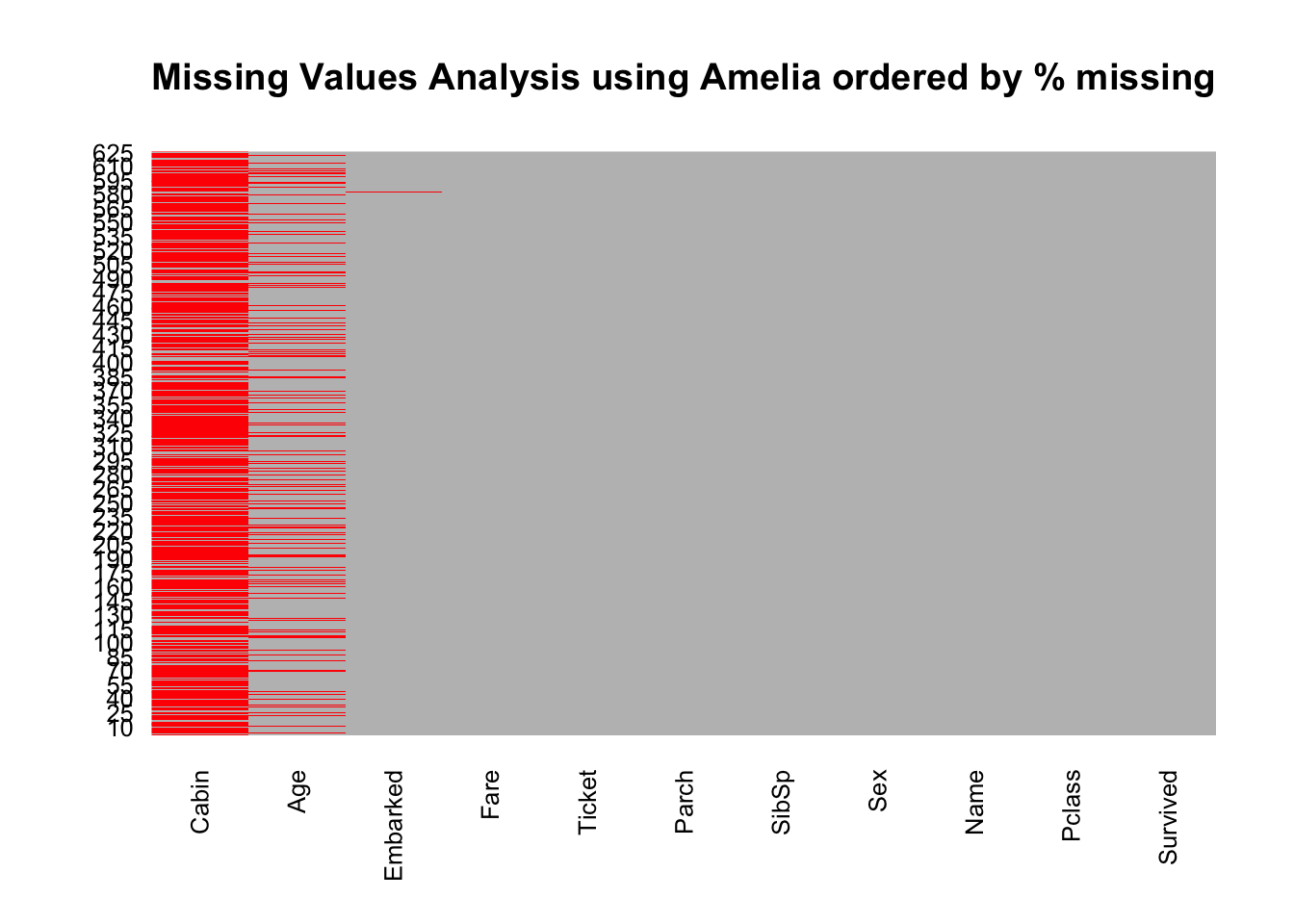``````#Missing cases (numbers):
map_int(train.raw,~sum(is.na(.x)))``````
``````## Survived   Pclass     Name      Sex      Age    SibSp    Parch   Ticket
##        0        0        0        0      117        0        0        0
##     Fare    Cabin Embarked
##        0      478        2``````

Cabin has a large number of missing values (77% missing). Imputing this variable may prove challenging or even useless. Age (19.9% missing) and Embarked (0.2%) missing are much more managable.

# EDA

The first step in the analysis is to explore the data numerically and graphically. I always split up my EDA investigation as follows:

• Target Variable
• Predictor Variables
• Univariate
• Bivariate
• Multivariate

This gives me a structured approach towards larger datasets. My professor at Northwestern taught me to always complete a thorough intimate numeric & graphical EDA on the data, no matter how large the data 1. Anscombe (1973) clearly shows the importance of graphical analyses.

## Target Variable

`Survived` is the response variable. As we can see, a large majority of the passengers did not survive the accident. The response variable is a False/True boolean variable. Thus, the analysis techniques used later will be those appropriate for classification problems.

``round(prop.table(table(train.raw\$Survived)),2)``
``````##
##     0.38     0.62``````

The classes are ~40:60 split which isn’t too bad. Probably don’t have to do any class balancing work.

## Predictor Variables

### Univariate & Bivariate

The first step is to look at every variable available. I prefer using the `ggplot2` framework for all the visuals.

#### Continuous Variables

• `Age` seems to have a bimodal distribution - very young children, and then directly young adults to mid-age persons. The 2nd mode is right skewed with no obvious outliers.

• `Fare` certainly shows many outliers beyond the ~\$200 level. A majority of the fares are <\$50, which makes sense since a majority of the travelers are bound to be in the 3rd passenger class.

``````p1 <- ggplot(data=train.raw,aes(x=Age))  + geom_histogram(aes(fill=Survived),bins = 40) + coord_flip()
p2 <- ggplot(data=train.raw,aes(x=Fare)) + geom_histogram(aes(fill=Survived),bins = 40) + coord_flip()
grid.arrange(p1,p2,nrow=1)``````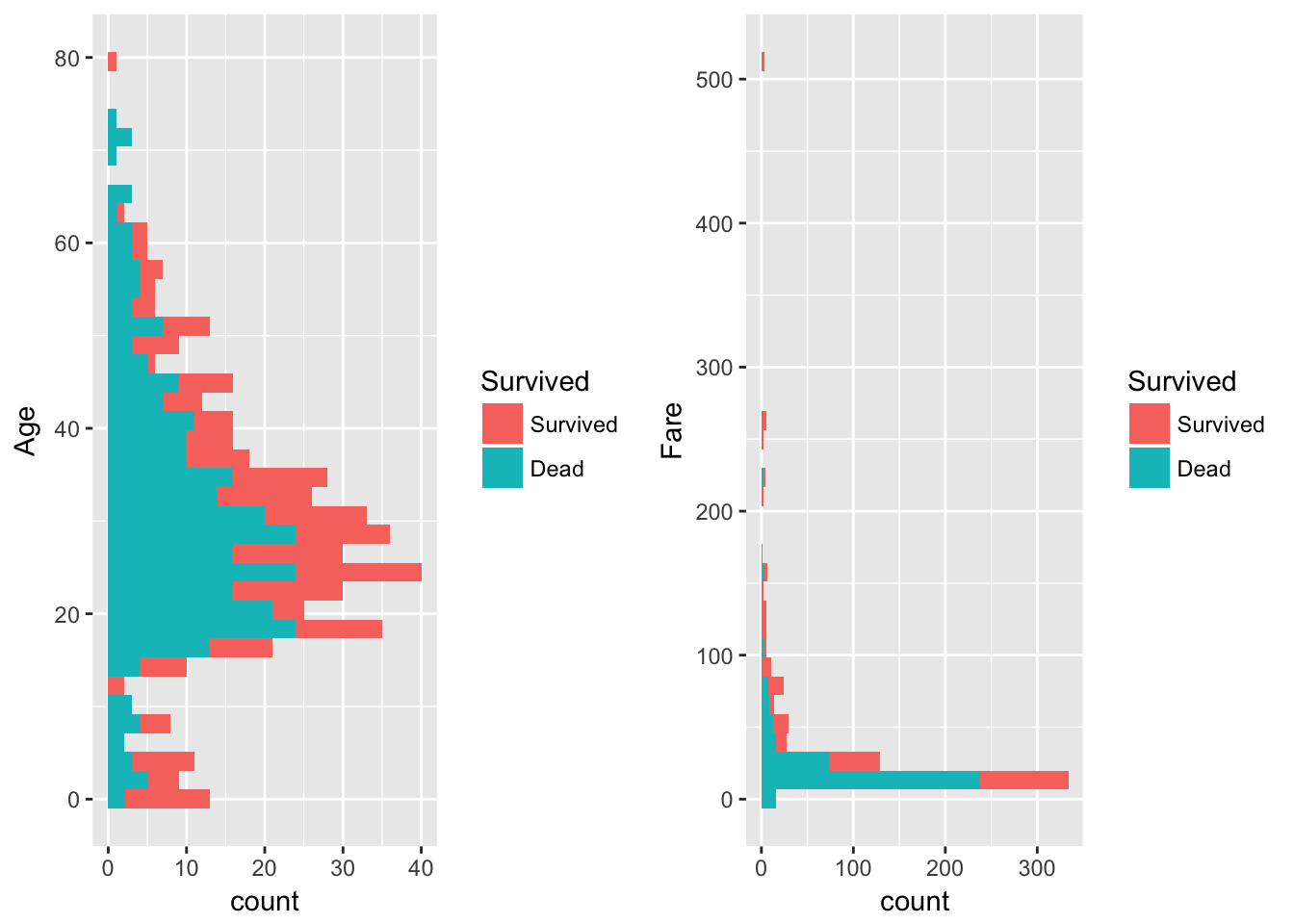As we can see, the median fare is \$14.5, the mean is \$32, but the max is \$512. We’ll investigate winzorising this variable in the latter part. Perhaps a transformation will also help?

``summary(train.raw\$Fare)``
``````##    Min. 1st Qu.  Median    Mean 3rd Qu.    Max.
##   0.000   7.925  14.500  33.710  31.000 512.329``````

#### Categorical Variables

A ggplot command is iterated over for the categorical variables.2

Key takeways for the categorical variables:

1. `Pclass`: If you were traveling 1st class, you have the highest chance of survival. Could be indicative of preferential treatment to those who paid more, a less politically correct class-stratified society, as well as the fact that the 1st class passengers had cabins at the very top of the ship.
2. `Pclass`: Persons traveling 3rd class had the highest fatality rate. 3rd class passengers had cabins deep in the ship. With the reasons give in (1), this could have contributed to the low survival rate.
3. `Sex`: Males have a very high fatality rate. Seems like the ‘women and children’ first policy was followed during evacuation.
4. `SibSp` & `Parch`: What’s interesting here is, for both these variables, at level 0, the fatality rate is higher. At levels 1+, the chances of survival are much better. Again, this could point to the ‘women and children’ policy being followed. (Or perhaps there weren’t as many families with children on board!)
5. `Embarked`: Southampton has a higher fatality rate than Cherbourg or Queenstown. A cross-tabulation between `Embarked` and `Pclass` shows that 72% of the 3rd class passengers and 89% of the 2nd class passengers boarded at Southampton. This jives with the observation that 2nd and 3rd class passengers have higher fatality rates.
``````get_legend<-function(myggplot){
tmp <- ggplot_gtable(ggplot_build(myggplot))
leg <- which(sapply(tmp\$grobs, function(x) x\$name) == "guide-box")
legend <- tmp\$grobs[[leg]]
return(legend)
}
p <- lapply(X = c('Pclass','Sex','SibSp','Parch','Embarked'),
FUN = function(x) ggplot(data = train.raw)+
aes_string(x=x,fill='Survived')+
geom_bar(position="dodge")+
theme(legend.position="none"))
legend <- get_legend(ggplot(data = train.raw,aes(x=Pclass,fill=Survived))+geom_bar())
grid.arrange(p[],p[],p[],p[],p[],
legend,layout_matrix = cbind(c(1,2,3),
c(4,5,3),
c(6,6,6)),
widths=c(3,3,1))``````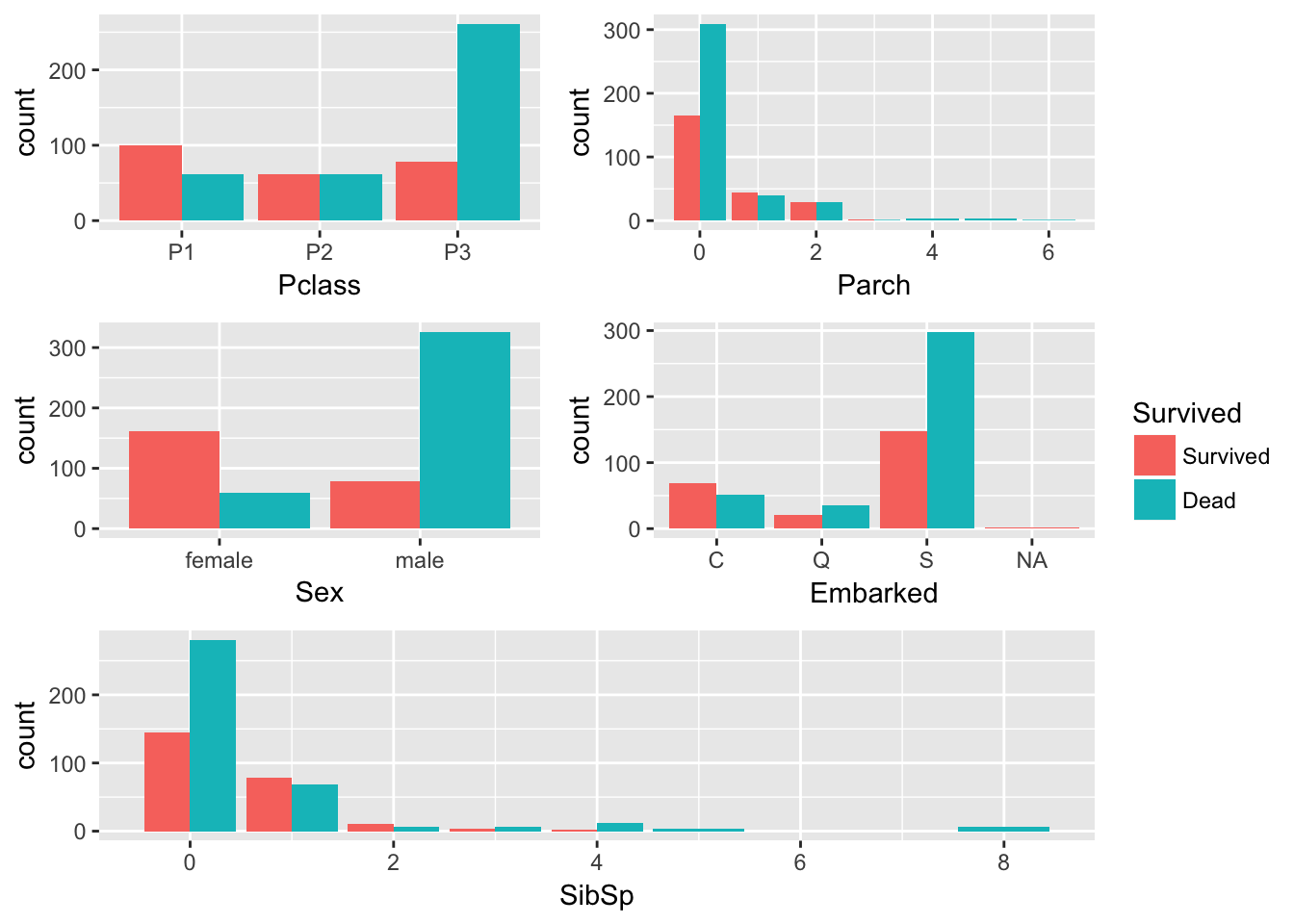### Multivariate Analyses

Grouped boxplots are a common method of comparing distributions grouped by categorical variables. I find beanplots to be excellent complementary plots to boxplots (and in some cases, even better). They’re a bit tricky to read at first - since they are so underutilized - but just through one plot, a wealth of information can be extracted.3

Here is a comparison of the same information between a boxplot and a beanplot. What can we infer from the bean plot better?

1. The beanplot allows us to visualize the density function of the parameter, in this case: Age. Furthermore, the length of each beanline is cumulative to the number of datapoints that exist. Rightaway, we can tell that Pclass=3 has the most data in the set, with sparser data at Pclass=1.
2. The mean values for 1st class is higher than that for 2nd and 3rd class. The distributions of deceased and survived for 1st class are fairly similar.
3. For 2nd and 3rd class, the survived data shows a bimodal distribution. Bumps at the 0-10 age show that children were evacuated first. This is also the reason the mean values for survived is lower.
4. For 2nd and 3rd class, the deceased data shows a fairly normal distribution.
5. The individual measurements (represented by black lines) represent each observation and help identify outliers much more easily than a boxplot does.
``````ggplot(train.raw,aes(y=Age,x=Pclass))+geom_boxplot(aes(fill=Survived))+theme_bw()
beanplot(Age~Survived*Pclass,side='b',train.raw,col=list('yellow','orange'),
border = c('yellow2','darkorange'),ll = 0.05,boxwex = .5,
main='Passenger survival by pclass and Age',xlab='Passenger Class',ylab='Age')
legend('topright', fill = c('yellow','orange'), legend = c("Dead", "Survived"),bty = 'n',cex = .8)``````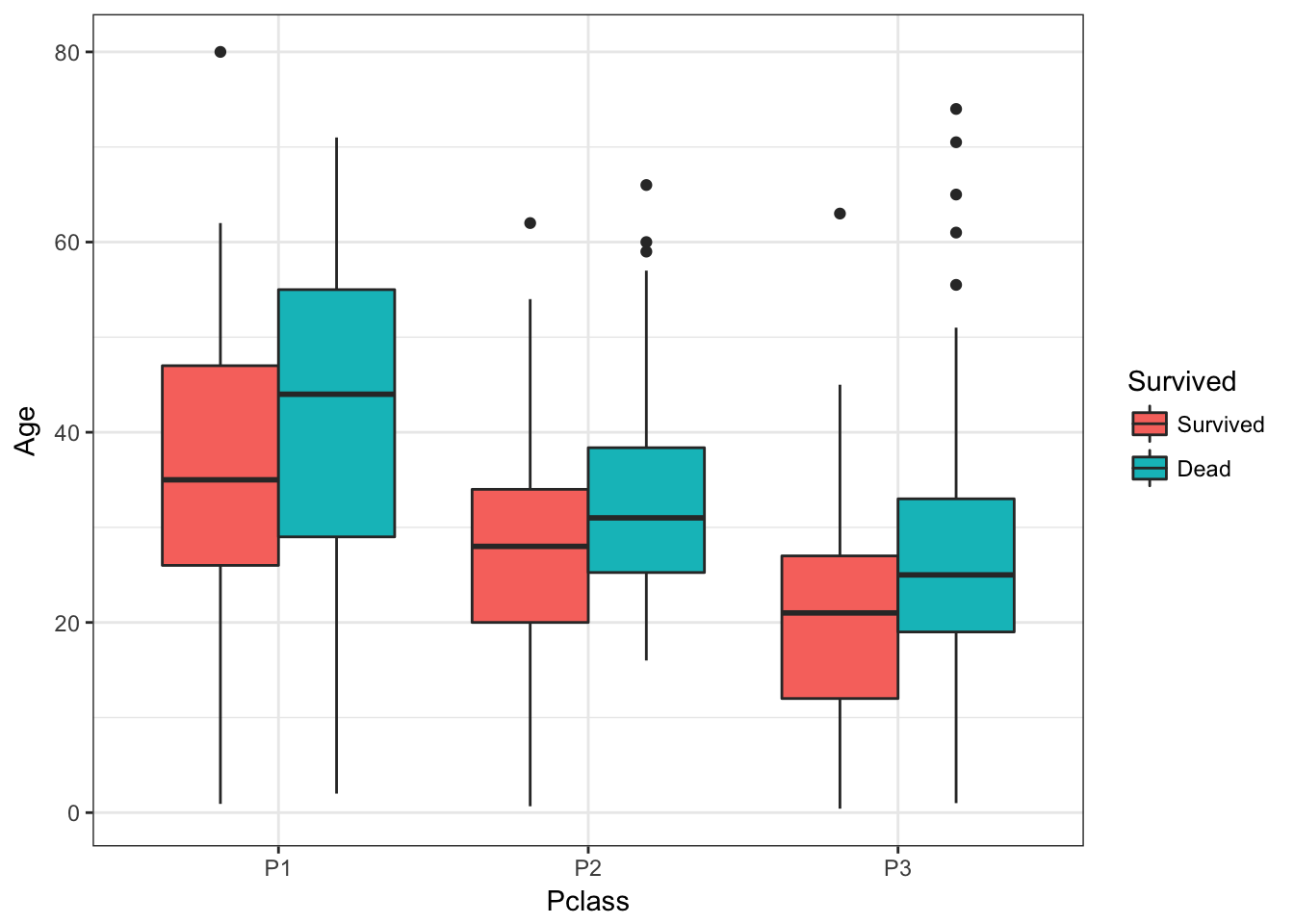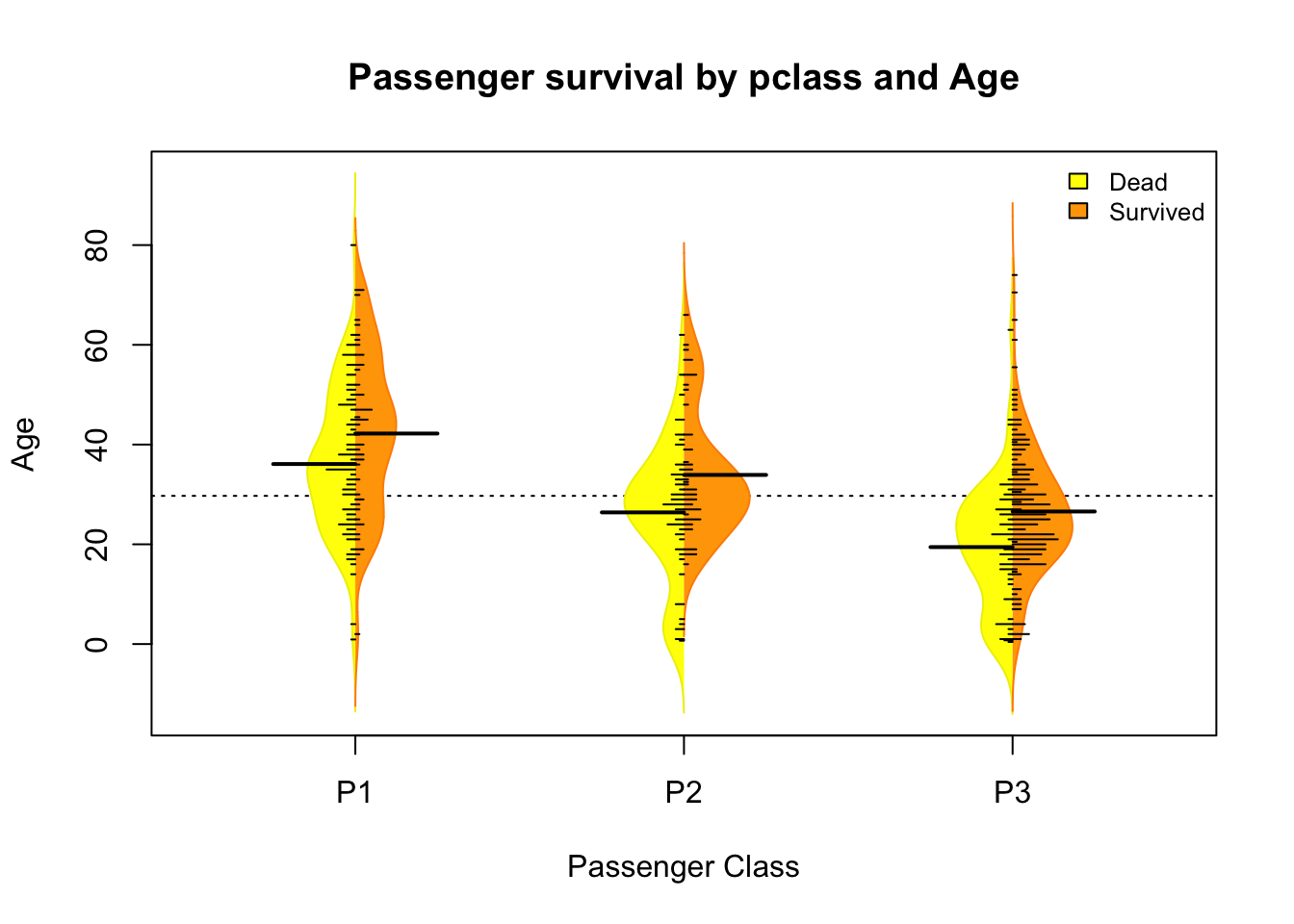A look into the `SibSp` and `Parch` variables shows something interesting. There are three regions one can identify:

• The probability of survival is minimal for number of parents/children aboard > 3.
• The probability of survival is minimal for number of siblings/spouses aboard > 3.
• For `SibSp`<=3 and `Parch`<=3, there are better chances for survival.

The grouping by `Pclass` reveals that all the large families were 3rd class travelers. Worse access to help… lowest chance for survival.

These could be simple rules either hard coded during model building: something along the lines of: IF (SibSp>3 OR Parch >3) THEN prediction = 0, or some derived variables can be created.

``````ggplot(train.raw,aes(y=SibSp,x=Parch))+
geom_jitter(aes(color=Survived,shape=Pclass))+
theme_bw()+
scale_shape(solid=F)+
geom_vline(xintercept = 3,color='darkred',lty=2)+
geom_hline(yintercept = 3,color='red',lty=2)``````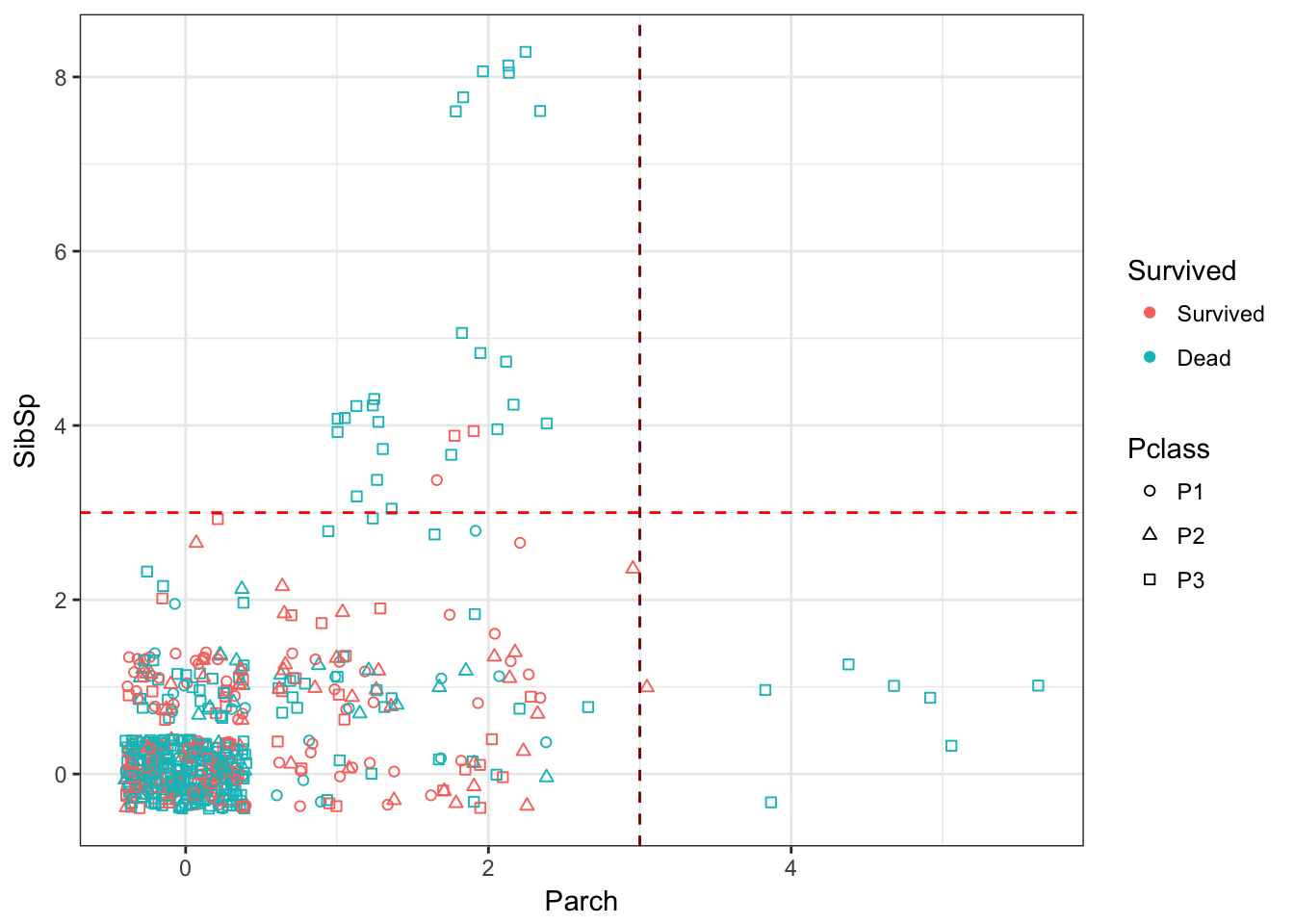Another excellent way to look at multivariate data is using alluvial plots. Not only are these plots intuitive to read and visually stiking, but they offer a lot of insight into the structure of the multivariate data as well.

``````train.raw %>%
mutate(Age_Group = case_when(
Age < 18 ~ 'Child',
Age >= 18 ~ 'Adult'
)) %>%
group_by(Survived, Sex, Pclass, Age_Group) %>%
summarise(N = n()) %>%
ungroup %>%
na.omit -> alluvial_table

alluvial(alluvial_table[,c(-5)],
freq = alluvial_table\$N,
cex = 0.8,
col=ifelse(alluvial_table\$Survived == "Survived", "blue", "forestgreen"))``````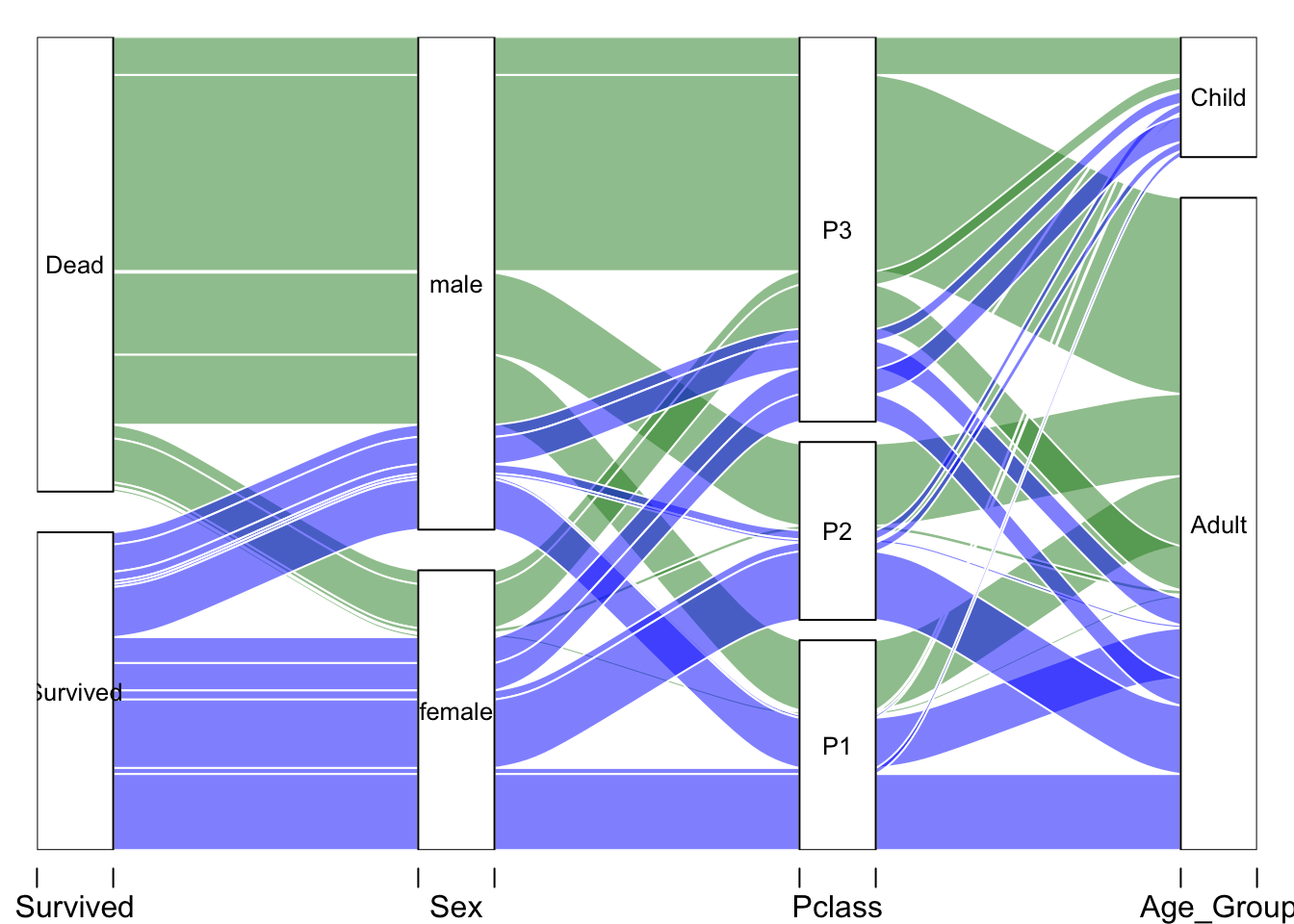# Data Preparation

## Missing Values Imputation

Starting with the easier one first:

Embarked: The largest portion of the passengers embared at Southhampton. I’m replacing the NAs with the same. First, I create a new imputed training dataset.

``summary(train.raw\$Embarked)``
``````##    C    Q    S NA's
##  120   57  446    2``````
``````train.imp <- train.raw
train.imp\$Embarked[is.na(train.imp\$Embarked)] <- 'S'``````

Names, Titles & Age: The names have titles embedded in the strings. I can extract these using regex. Master, Miss, Mr and Mrs are the most popular - no surprise there, with lots of other titles. Here’s the distribution of the titles by age. These can be used to impute the missing age values.

``````train.imp\$title <- str_extract(pattern = '[a-zA-Z]+(?=\\.)',string = train.imp\$Name)
train.imp\$title <- as.factor(train.imp\$title)
ggplot(train.imp,aes(x=title,y=Age))+
geom_jitter(shape=21,alpha=.6,col='blue')+
stat_summary(aes(y = Age,group=1), fun.y=median, colour="red", geom="point",group=1)+
theme_bw()+
theme(axis.text.x = element_text(angle = 45, hjust = 1),legend.position="none")+
labs(caption='red points are median values')``````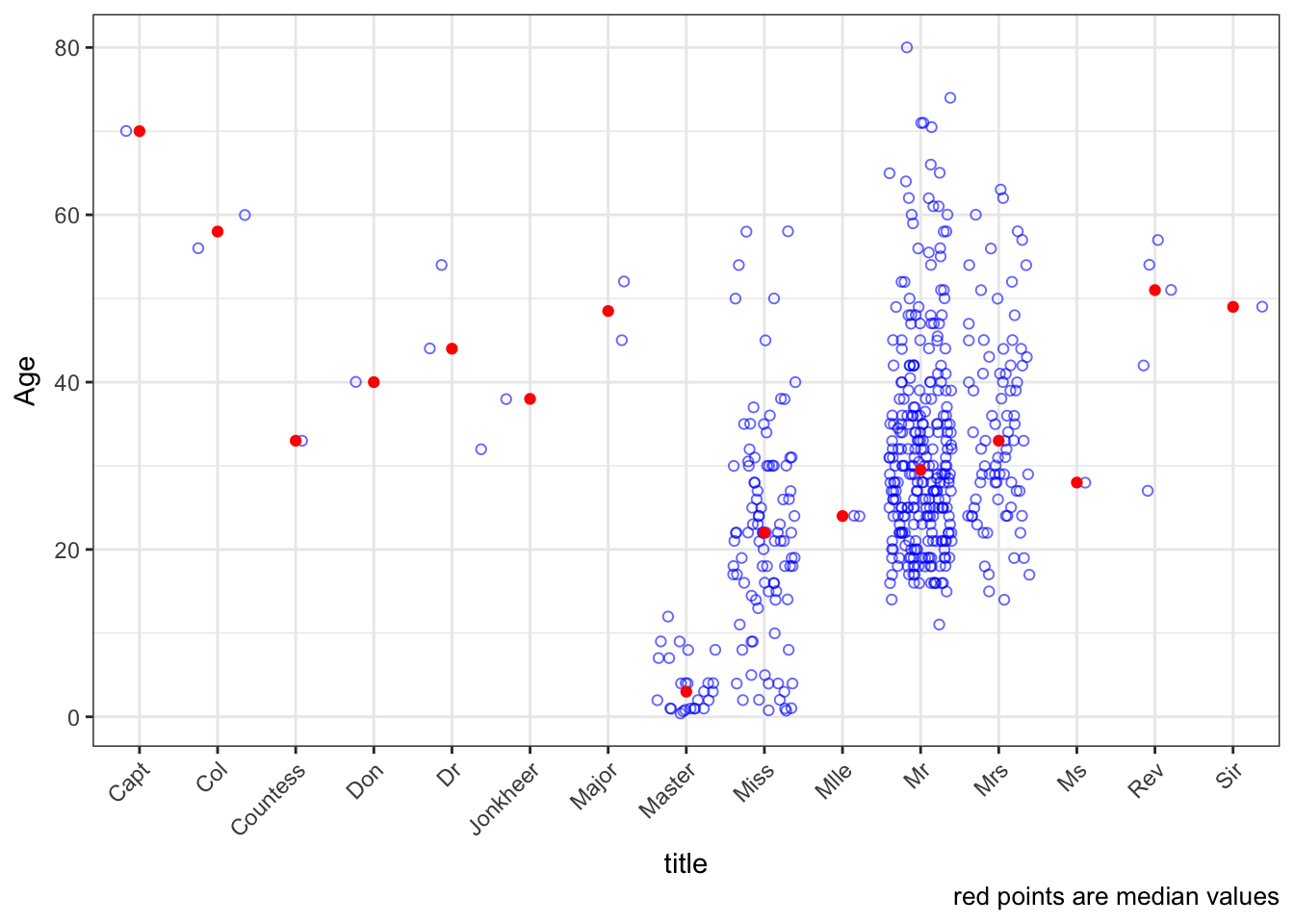Grouping similar titles together, I’ve kept a few titles - Officer, Royalty, Mr, Mrs and Miss.

``````train.imp\$title <- as.character(train.imp\$title)
train.imp\$title[train.imp\$title %in% c('Capt','Col','Major')] <- 'Officer'
train.imp\$title[train.imp\$title %in% c('Don','Dr','Rev','Sir','Jonkheer','Countess','Lady','Dona')] <- 'Royalty'
train.imp\$title[train.imp\$title %in% c('Mrs','Mme')] <- 'Mrs'
train.imp\$title[train.imp\$title %in% c('Ms','Mlle')] <- 'Miss'
train.imp\$title <- as.factor(train.imp\$title)
ggplot(train.imp,aes(x=title,y=Age))+
geom_jitter(color='blue',shape=21,alpha=.7)+
stat_summary(aes(y = Age,group=1), fun.y=median, colour="red", geom="point",group=1)+
theme_bw()+
theme(axis.text.x = element_text(angle = 45, hjust = 1))+
labs(caption='red points are median values')``````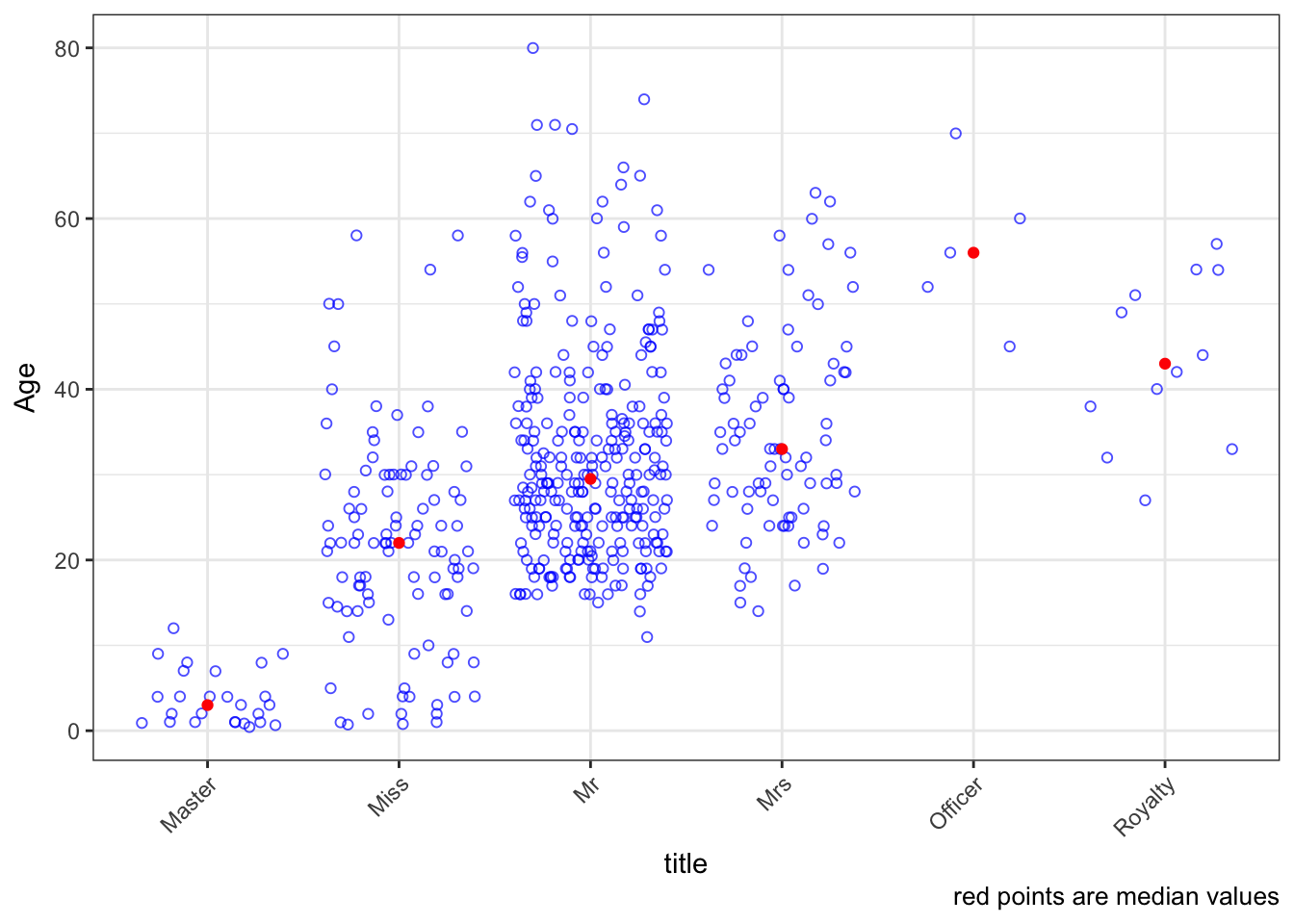Now for the missing Age values. I’m trying out two strategies to impute age, just for kicks. First, a regression tree using the `rpart` method. 5-repeat 10-fold cross validation across a tuning grid of 20 values of `maxdepth`. RMSE stablizes at a depth of 14, with a value of 12.2.

``````age.predictors <- train.imp %>%
dplyr::select(-Survived,-Cabin,-Ticket,-Name) %>%
dplyr::filter(complete.cases(.))
ctrl <- trainControl(method = "repeatedcv",
repeats = 5)
rpartGrid <- data.frame(maxdepth = seq(2,10,1))
rpartFit_ageimputation <- train(x=age.predictors[,-3],
y=age.predictors\$Age,
method='rpart2',
trControl = ctrl,
tuneGrid = rpartGrid
)
rpartFit_ageimputation``````
``````## CART
##
## 508 samples
##   7 predictor
##
## No pre-processing
## Resampling: Cross-Validated (10 fold, repeated 5 times)
## Summary of sample sizes: 457, 457, 457, 457, 457, 457, ...
## Resampling results across tuning parameters:
##
##   maxdepth  RMSE      Rsquared   MAE
##    2        12.02414  0.3171031  9.443687
##    3        11.30498  0.3985131  8.707856
##    4        11.42463  0.3882499  8.782511
##    5        11.27085  0.4038018  8.639549
##    6        11.39825  0.3930011  8.720958
##    7        11.43177  0.3890118  8.744528
##    8        11.47797  0.3851413  8.783542
##    9        11.48005  0.3848860  8.783870
##   10        11.48005  0.3848860  8.783870
##
## RMSE was used to select the optimal model using the smallest value.
## The final value used for the model was maxdepth = 5.``````
``````plot(rpartFit_ageimputation)
rpart.plot::rpart.plot(rpartFit_ageimputation\$finalModel, extra=101, box.palette="GnBu")
save(rpartFit_ageimputation,file = 'rpartFit_ageimputation')``````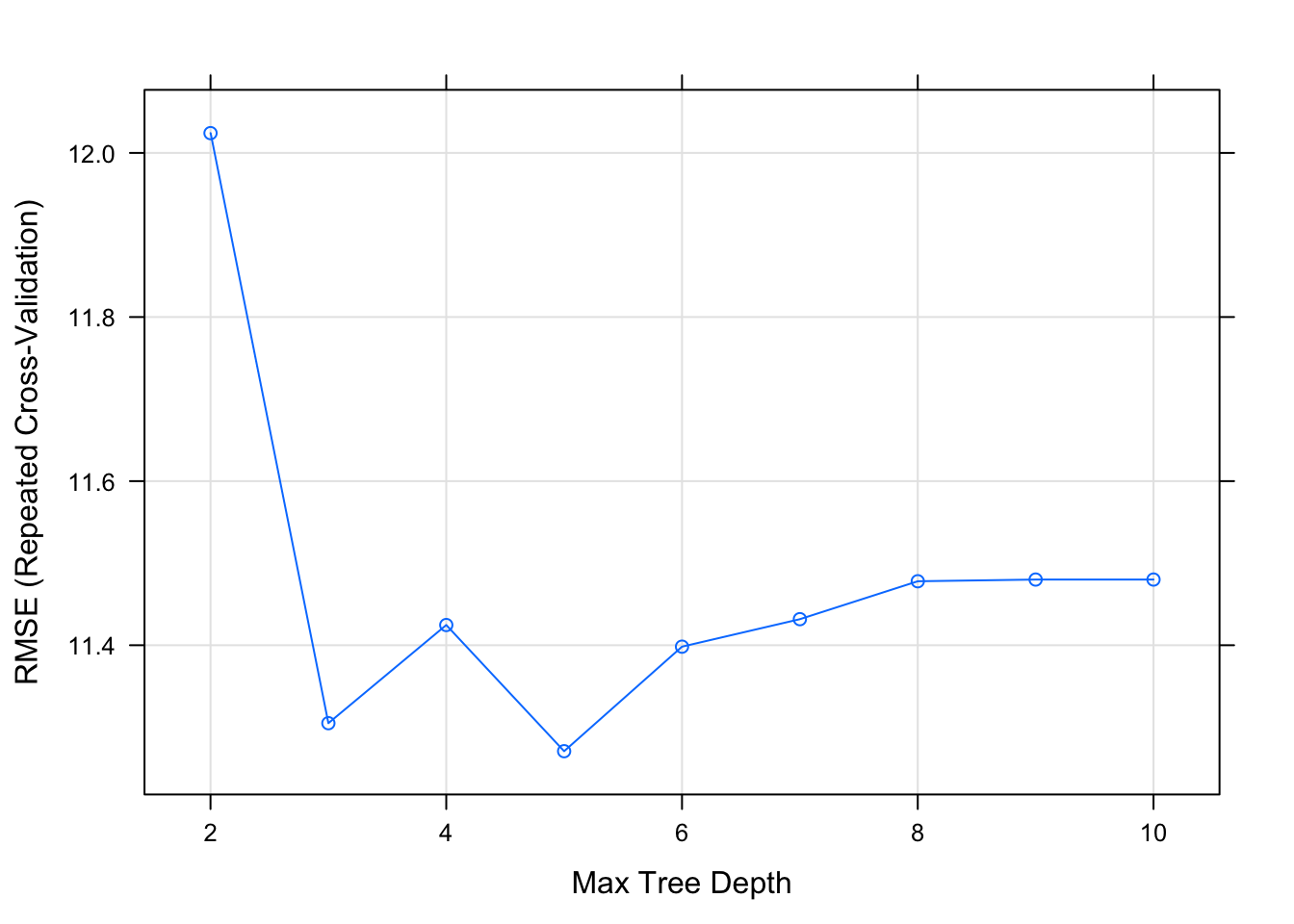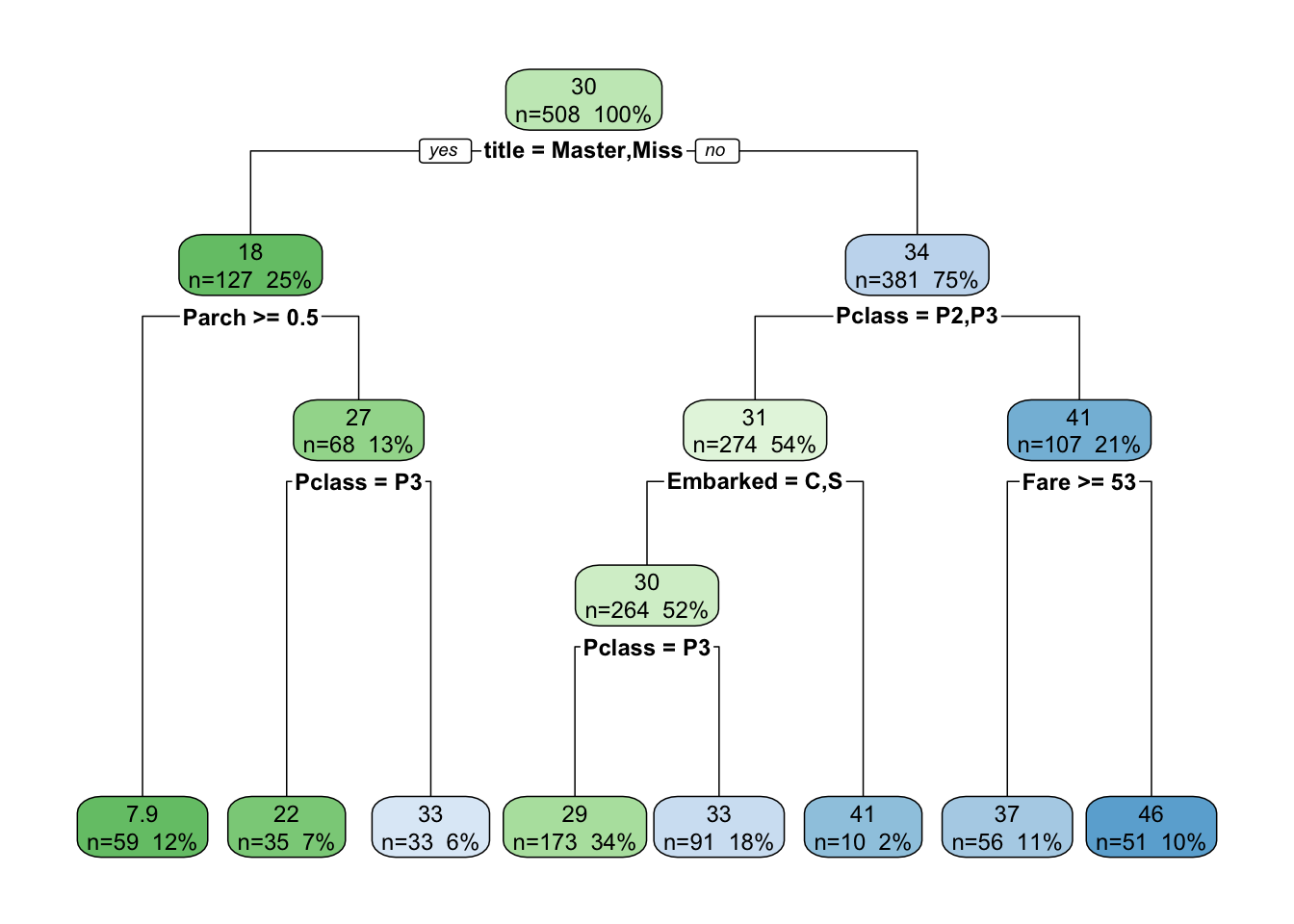I’m going to use the randomForest model. Using the `predict.train()` to predict values of age and plug them back into the imputed data. You can see the blue points which are the imputed values of `Age`. What I noticed is that for all the titles, the imputed Age value seems to be distributed fairly well, except Master. For Master, the three imputed are definitely outliers. I’m going to force these to the median Age.

``````missing_age <- is.na(train.imp\$Age)
age.predicted <- predict(rpartFit_ageimputation, newdata = train.imp[missing_age,])
train.imp[missing_age,'Age'] <- age.predicted

train.imp %>%
mutate(Age_Imputed = missing_age) %>%
ggplot(aes(x=title,y=Age))+
stat_summary(aes(y = Age,group=1), fun.y=median, colour="red", geom="point",group=1)+
geom_jitter(aes(y=Age,col=Age_Imputed,shape=Age_Imputed))+
theme_bw()+
theme(axis.text.x = element_text(angle = 45, hjust = 1),legend.position="none")+
labs(caption='green points are imputed values')``````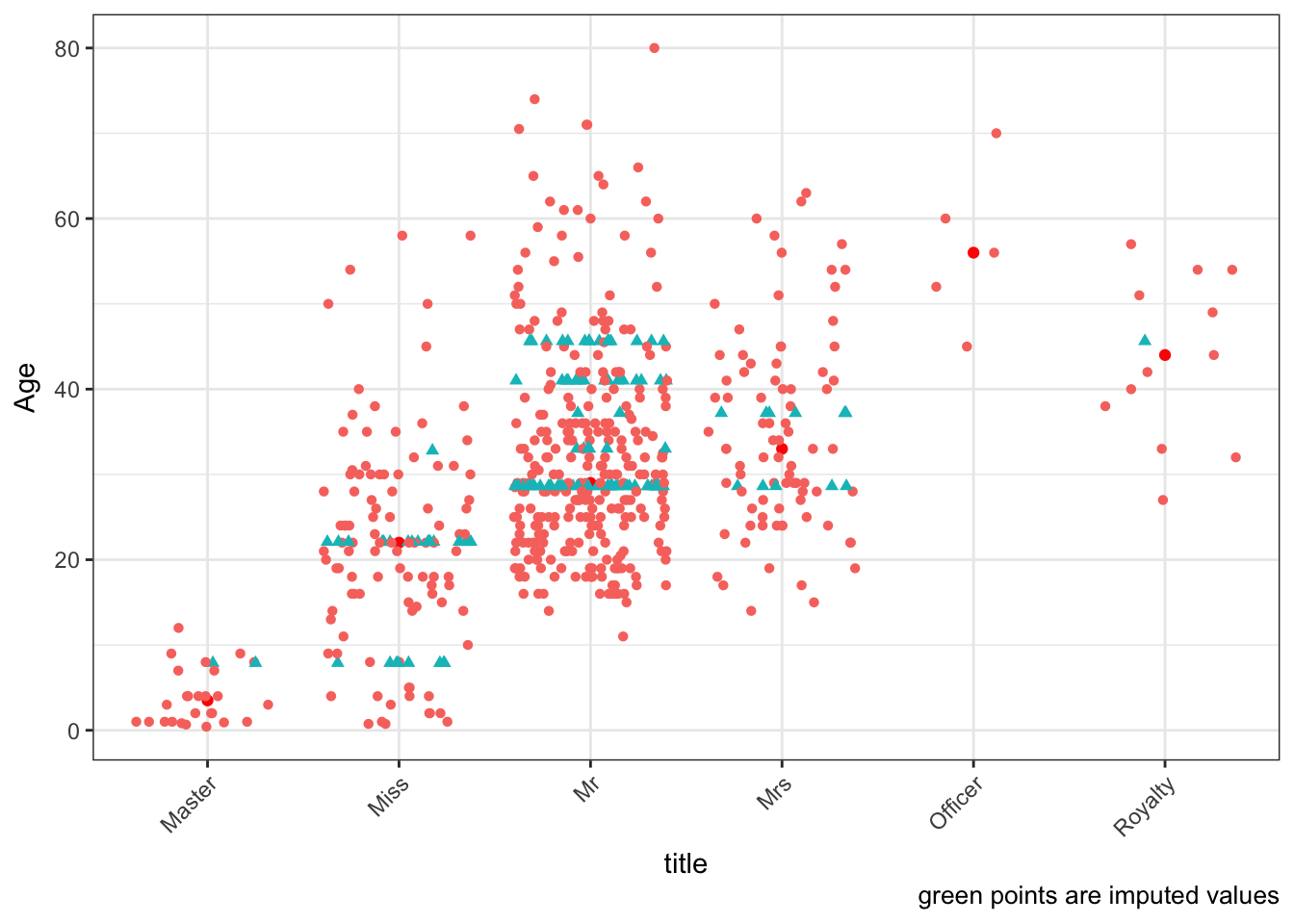## Derived Variables

Child?: Trying out two engineered variables here - is the passenger a child or not? Using Age=18 as a threshold.

``````train.imp\$child <- 0
train.imp\$child[train.imp\$Age<18] <- 1``````

Really old?: Really older folks would get priority perhaps. Creating a categorical variables for this conditions.

``train.imp\$Seniors <- ifelse(train.imp\$Age>60,1,0)``

Family related: Let’s also create some variables that talk about family sizes. What’s the total family size – continous variable `TotalFam`. Is the person single, part of a couple or a large family? Three categorical variables for these.

``````train.imp\$TotalFam <- train.imp\$SibSp + train.imp\$Parch + 1
train.imp\$LargeFamily <- ifelse(train.imp\$TotalFam>4,1,0)
train.imp\$Name <- NULL``````

Cabin related: Extracting the cabin alphabet and number from the cabin variable. Since the cabin numbers could be ordered from left to right or top to bottom on the boat, perhaps only the 1st digit is significant. Also, some folks have more than 1 cabin. Wonder if that’s important. Since lots of unknowns in the `Cabin` variable, all NA values are replaced by ‘U’. Refering to the deck diagram, the topmost decks are A and B, which are closest to the lifeboats. Perhaps that’s important too. Here, I create a bunch of categorical variables based off the original `Cabin`, and then remove it from the dataset.

``````train.imp\$CabinMissing <- as.numeric(is.na(train.raw\$Cabin))

train.imp\$CabinCode <- map_chr(train.raw\$Cabin,~str_split(string = .x,pattern = '')[])
train.imp\$CabinCode[is.na(train.imp\$CabinCode)] <- 'U'
train.imp\$CabinCode <- as.factor(train.imp\$CabinCode)

train.imp\$CabinNum <- as.numeric(map_chr(train.raw\$Cabin,~str_split(string = .x,pattern = '[a-zA-Z]')[]))
train.imp\$CabinNum <- map_int(train.imp\$CabinNum, ~as.integer(str_split(.x,pattern = '',simplify = T)))
train.imp\$CabinNum[is.na(train.imp\$CabinNum)] <- 0

train.imp\$TopDeck <- ifelse(train.imp\$CabinCode %in% c('A','B'),1,0)
train.imp\$MidDeck <- ifelse(train.imp\$CabinCode %in% c('C','D'),1,0)
train.imp\$LowerDeck <- ifelse(train.imp\$TopDeck==0 & train.imp\$MidDeck==0 ,1,0)

train.imp\$NumberofCabins <- map_int(train.raw\$Cabin,~str_split(string = .x,pattern = ' ')[] %>% length)
train.imp\$Cabin <- NULL``````

Ticket: Lastly, the `ticket` variable. I’m not sure what to make of it, so I’m keeping it for now, after cleaning it up a bit. A majority (80%) of the rows have unique (one) ticket. 14% rows have a duplicate ticket, perhaps indicating a family. A small number of rows have 3+ duplicates of the tickets.

``train.imp\$Ticket %>% table() %>% as.numeric() %>% table()``
``````## .
##   1   2   3   4   5   6   7
## 430  60  15   3   1   1   1``````

There seems to be a bit of a pattern here. Tickets starting with 1 are mostly 1st class, those starting with 2 are 2nd class, and 3 - 3rd class. But, I feel it’s a very loose association.

``train.imp %>% group_by(Pclass) %>% dplyr::select(Ticket,Pclass) %>% sample_n(5)``
``````## # A tibble: 15 x 2
## # Groups:   Pclass 
##    Ticket       Pclass
##    <chr>        <fct>
##  1 112059       P1
##  2 PC 17599     P1
##  3 17474        P1
##  4 113503       P1
##  5 36963        P1
##  6 248731       P2
##  7 237668       P2
##  8 W./C. 14263  P2
##  9 S.O.C. 14879 P2
## 10 29011        P2
## 11 LINE         P3
## 12 350047       P3
## 13 348123       P3
## 14 371110       P3
## 15 2627         P3``````

What I’m going to do is clean up the columns (remove special characters, spaces etc), then split the `Ticket` column into four: `TicketChar`, `TicketNum`,`TicketNumLength`, `TicketNumStart`. (Upon running the script a few times, I’ve decided to get rid of `TicketNum`, but I’m commenting the code for future ref). The `TicketChar` variable as this distribution:

``````train.imp %<>%
mutate(
Ticket = str_to_upper(Ticket) %>%
str_replace_all(pattern = regex(pattern = '[.\\/]'),replacement = ''),
TicketNum = str_extract(Ticket,pattern = regex('([0-9]){3,}')),
TicketNumStart = map_int(TicketNum,~as.integer(str_split(.x,pattern = '',simplify = T))),
TicketNumLen = map_int(TicketNum,~dim(str_split(.x,pattern = '',simplify = T))),
TicketChar = str_extract(Ticket,pattern = regex('^[a-zA-Z/\\.]+'))
) %>%
mutate(
TicketChar = map_chr(.x=TicketChar,
.f=~str_split(string=.x, pattern = '',simplify = T))
) %>%
mutate(
TicketChar = ifelse(is.na(TicketChar),'U',TicketChar),
TicketNumStart = ifelse(is.na(TicketNumStart),0,TicketNumStart),
TicketNumLen = ifelse(is.na(TicketNumLen),0,TicketNumLen),
) %>%
mutate(
TicketChar = as.factor(TicketChar),
TicketNumStart = factor(TicketNumStart,levels = seq(0,9,1)),
TicketNumLen = as.factor(TicketNumLen)
)
train.imp\$Ticket <- NULL
train.imp\$TicketNum <- NULL
table(train.imp\$TicketChar,dnn ='TicketChar')``````
``````## TicketChar
##   A   C   F   L   P   S   U   W
##  15  36   3   3  52  48 461   7``````
``table(train.imp\$TicketNumLen,dnn='TicketNumLen')``
``````## TicketNumLen
##   1   3   4   5   6   7
##   5   5 112 171 298  34``````
``table(train.imp\$TicketNumStart,dnn='TicketNumStart')``
``````## TicketNumStart
##   0   1   2   3   4   5   6   7   8   9
##   5 171 150 256  11   7   9  12   1   3``````
``table(train.imp\$NumberofCabins)``
``````##
##   1   2   3   4
## 606  12   5   2``````

## Final Data Review

The dataset is now prepared for modeling. Here’s a quick review of the data so far. 23 variables in total.

``train.imp %>% glimpse()``
``````## Observations: 625
## Variables: 23
## \$ Pclass         <fct> P3, P1, P3, P3, P3, P3, P2, P3, P1, P3, P3, P3,...
## \$ Sex            <fct> male, female, female, male, male, male, female,...
## \$ Age            <dbl> 22.00000, 38.00000, 26.00000, 35.00000, 41.0000...
## \$ SibSp          <int> 1, 1, 0, 0, 0, 3, 1, 1, 0, 1, 0, 4, 0, 1, 0, 0,...
## \$ Parch          <int> 0, 0, 0, 0, 0, 1, 0, 1, 0, 5, 0, 1, 0, 0, 0, 0,...
## \$ Fare           <dbl> 7.2500, 71.2833, 7.9250, 8.0500, 8.4583, 21.075...
## \$ Embarked       <fct> S, C, S, S, Q, S, C, S, S, S, S, Q, S, S, S, Q,...
## \$ title          <fct> Mr, Mrs, Miss, Mr, Mr, Master, Mrs, Miss, Miss,...
## \$ child          <dbl> 0, 0, 0, 0, 0, 1, 1, 1, 0, 0, 1, 1, 0, 0, 0, 1,...
## \$ Seniors        <dbl> 0, 0, 0, 0, 0, 0, 0, 0, 0, 0, 0, 0, 0, 0, 0, 0,...
## \$ TotalFam       <dbl> 2, 2, 1, 1, 1, 5, 2, 3, 1, 7, 1, 6, 1, 2, 1, 1,...
## \$ LargeFamily    <dbl> 0, 0, 0, 0, 0, 1, 0, 0, 0, 1, 0, 1, 0, 0, 0, 0,...
## \$ CabinMissing   <dbl> 1, 0, 1, 1, 1, 1, 1, 0, 0, 1, 1, 1, 1, 1, 1, 1,...
## \$ CabinCode      <fct> U, C, U, U, U, U, U, G, C, U, U, U, U, U, U, U,...
## \$ CabinNum       <dbl> 0, 8, 0, 0, 0, 0, 0, 6, 1, 0, 0, 0, 0, 0, 0, 0,...
## \$ TopDeck        <dbl> 0, 0, 0, 0, 0, 0, 0, 0, 0, 0, 0, 0, 0, 0, 0, 0,...
## \$ MidDeck        <dbl> 0, 1, 0, 0, 0, 0, 0, 0, 1, 0, 0, 0, 0, 0, 0, 0,...
## \$ LowerDeck      <dbl> 1, 0, 1, 1, 1, 1, 1, 1, 0, 1, 1, 1, 1, 1, 1, 1,...
## \$ NumberofCabins <int> 1, 1, 1, 1, 1, 1, 1, 1, 1, 1, 1, 1, 1, 1, 1, 1,...
## \$ TicketNumStart <fct> 2, 1, 3, 3, 3, 3, 2, 9, 1, 3, 3, 3, 2, 3, 2, 3,...
## \$ TicketNumLen   <fct> 5, 5, 7, 6, 6, 6, 6, 4, 6, 6, 6, 6, 6, 6, 6, 6,...
## \$ TicketChar     <fct> A, P, S, U, U, U, U, P, U, U, U, U, U, U, U, U,...``````

# Modeling

I’m experimenting with a few modeling techniques, mainly xgboost, penalized models using glmnet, kNN, SVM with RBF, C5.0 and Avg NN. I’ve tried to select models with different structures and different underlying architecture. This gives the highest diversity during the initial modeling approach. I’ve implemented all these models using caret which I find an absolutely indispensible toolkit to prep, build, tune and explore numerous models using very few lines of code.

For all models, I’m using a 5-repeat 10-fold cross validation technique on the training dataset. Tuning parameter searches (aka hypertuning) is performed using the `tuneGrid` parameter in the `train()` call. The best model is selected using the AUC of the ROC. Here are the models and a few intermediate results for each model. At the end, I’ve compared the performance of all the models together.

## Extreme Gradient Boosting

The renowned and popular `xgboost` package via `caret`. `ctrl` is the training control object which specifies the resampling method selected. The `grid` object helps perform hyperparameter tuning.

``````ctrl <- trainControl(method = "repeatedcv",
repeats = 5,
verboseIter = F,
classProbs = TRUE,
summaryFunction = twoClassSummary,
# sampling = 'down',
savePredictions = T
)
xgbGrid <- expand.grid(
nrounds=seq(14,24,2),
max_depth=seq(2,8,2),
eta=c(0.1, 0.2, 0.3),
gamma=1,
colsample_bytree=1,
min_child_weight=1,
subsample=1
)
xgbFit <- train(
Survived~.,
train.imp,
method = 'xgbTree',
trControl = ctrl,
tuneGrid = xgbGrid
)``````
``````## Warning in train.default(x, y, weights = w, ...): The metric "Accuracy" was
## not in the result set. ROC will be used instead.``````
``save(xgbFit,file = 'xgbFit')``

What we can see:

• Run times are extremely fast
• The final values used for the model were nrounds = 20, max_depth = 2, eta = 0.2. Perhaps increasing the boosting iterations might help, but I’m stopping here.
• Feature importance plots show the derived features ‘Senior’ and ‘TopDeck’ add value.
• The PDF of AUC ROC shows a bi-modal distribution (slightly). I noticed this for the other models as well. I wonder what that indicates?
• Histograms of the probability plots look somewhat as expected, with a strong peak at <0.2 for ‘Survived’ and a strong peak at >0.8 for ‘Dead’. What I don’t like is how it doesn’t seem to tail off at either end, i.e. ~20% of the data in either category is strongly misclassified.
``print(xgbFit,details = F)``
``````## eXtreme Gradient Boosting
##
## 625 samples
##  22 predictor
##   2 classes: 'Survived', 'Dead'
##
## No pre-processing
## Resampling: Cross-Validated (10 fold, repeated 5 times)
## Summary of sample sizes: 563, 562, 562, 562, 563, 563, ...
## Resampling results across tuning parameters:
##
##   eta  max_depth  nrounds  ROC        Sens       Spec
##   0.1  2          14       0.8735099  0.7158333  0.8722132
##   0.1  2          16       0.8742786  0.7125000  0.8742915
##   0.1  2          18       0.8750402  0.7166667  0.8763698
##   0.1  2          20       0.8745490  0.7208333  0.8800000
##   0.1  2          22       0.8749362  0.7166667  0.8800135
##   0.1  2          24       0.8748937  0.7216667  0.8815655
##   0.1  4          14       0.8808738  0.7475000  0.8903104
##   0.1  4          16       0.8825793  0.7483333  0.8939271
##   0.1  4          18       0.8826153  0.7500000  0.8955196
##   0.1  4          20       0.8826400  0.7500000  0.8986235
##   0.1  4          22       0.8822548  0.7525000  0.8986505
##   0.1  4          24       0.8814086  0.7458333  0.8975978
##   0.1  6          14       0.8788329  0.7383333  0.8773819
##   0.1  6          16       0.8785664  0.7450000  0.8799460
##   0.1  6          18       0.8797321  0.7500000  0.8799730
##   0.1  6          20       0.8799963  0.7483333  0.8815385
##   0.1  6          22       0.8813048  0.7508333  0.8841296
##   0.1  6          24       0.8809042  0.7483333  0.8851687
##   0.1  8          14       0.8777050  0.7416667  0.8852227
##   0.1  8          16       0.8794582  0.7466667  0.8867746
##   0.1  8          18       0.8814659  0.7516667  0.8847099
##   0.1  8          20       0.8821058  0.7525000  0.8846964
##   0.1  8          22       0.8827522  0.7508333  0.8873144
##   0.1  8          24       0.8821356  0.7508333  0.8888799
##   0.2  2          14       0.8766281  0.7116667  0.8800000
##   0.2  2          16       0.8764803  0.7091667  0.8815520
##   0.2  2          18       0.8780986  0.7100000  0.8810661
##   0.2  2          20       0.8786030  0.7125000  0.8805533
##   0.2  2          22       0.8793396  0.7225000  0.8784480
##   0.2  2          24       0.8792088  0.7208333  0.8784615
##   0.2  4          14       0.8801664  0.7458333  0.8949528
##   0.2  4          16       0.8812129  0.7458333  0.8944669
##   0.2  4          18       0.8828281  0.7483333  0.8949798
##   0.2  4          20       0.8818756  0.7508333  0.8949663
##   0.2  4          22       0.8821876  0.7491667  0.8970715
##   0.2  4          24       0.8829254  0.7491667  0.8965317
##   0.2  6          14       0.8793848  0.7391667  0.8877058
##   0.2  6          16       0.8809202  0.7425000  0.8918893
##   0.2  6          18       0.8803273  0.7416667  0.8918893
##   0.2  6          20       0.8814170  0.7400000  0.8924426
##   0.2  6          22       0.8810554  0.7433333  0.8945074
##   0.2  6          24       0.8813939  0.7425000  0.8934413
##   0.2  8          14       0.8802252  0.7441667  0.8893117
##   0.2  8          16       0.8795254  0.7516667  0.8882996
##   0.2  8          18       0.8792850  0.7541667  0.8877868
##   0.2  8          20       0.8800042  0.7500000  0.8882726
##   0.2  8          22       0.8795249  0.7516667  0.8904049
##   0.2  8          24       0.8799564  0.7566667  0.8893387
##   0.3  2          14       0.8777637  0.7216667  0.8769231
##   0.3  2          16       0.8778874  0.7291667  0.8753576
##   0.3  2          18       0.8765267  0.7358333  0.8763833
##   0.3  2          20       0.8767673  0.7366667  0.8747908
##   0.3  2          22       0.8777370  0.7400000  0.8753171
##   0.3  2          24       0.8788827  0.7483333  0.8784480
##   0.3  4          14       0.8811111  0.7500000  0.8960189
##   0.3  4          16       0.8822934  0.7533333  0.8980972
##   0.3  4          18       0.8825172  0.7483333  0.8965182
##   0.3  4          20       0.8824303  0.7516667  0.8929015
##   0.3  4          22       0.8825464  0.7525000  0.8928745
##   0.3  4          24       0.8826560  0.7516667  0.8934143
##   0.3  6          14       0.8790562  0.7416667  0.8929690
##   0.3  6          16       0.8802716  0.7475000  0.8924696
##   0.3  6          18       0.8801054  0.7516667  0.8924561
##   0.3  6          20       0.8796410  0.7500000  0.8914170
##   0.3  6          22       0.8801425  0.7500000  0.8929960
##   0.3  6          24       0.8803093  0.7500000  0.8935223
##   0.3  8          14       0.8820339  0.7566667  0.8862213
##   0.3  8          16       0.8824120  0.7516667  0.8893657
##   0.3  8          18       0.8828990  0.7541667  0.8888664
##   0.3  8          20       0.8821952  0.7558333  0.8878408
##   0.3  8          22       0.8831267  0.7525000  0.8878677
##   0.3  8          24       0.8830929  0.7533333  0.8868151
##
## Tuning parameter 'gamma' was held constant at a value of 1
##  1
## Tuning parameter 'min_child_weight' was held constant at a value of
##  1
## Tuning parameter 'subsample' was held constant at a value of 1
## ROC was used to select the optimal model using the largest value.
## The final values used for the model were nrounds = 22, max_depth = 8,
##  eta = 0.3, gamma = 1, colsample_bytree = 1, min_child_weight = 1
##  and subsample = 1.``````
``````plot(xgbFit)
xgb.importance(feature_names = colnames(train.imp),
model = xgbFit\$finalModel) %>%
xgb.ggplot.importance()
densityplot(xgbFit,pch='|')
predict(xgbFit,type = 'prob') -> train.Probs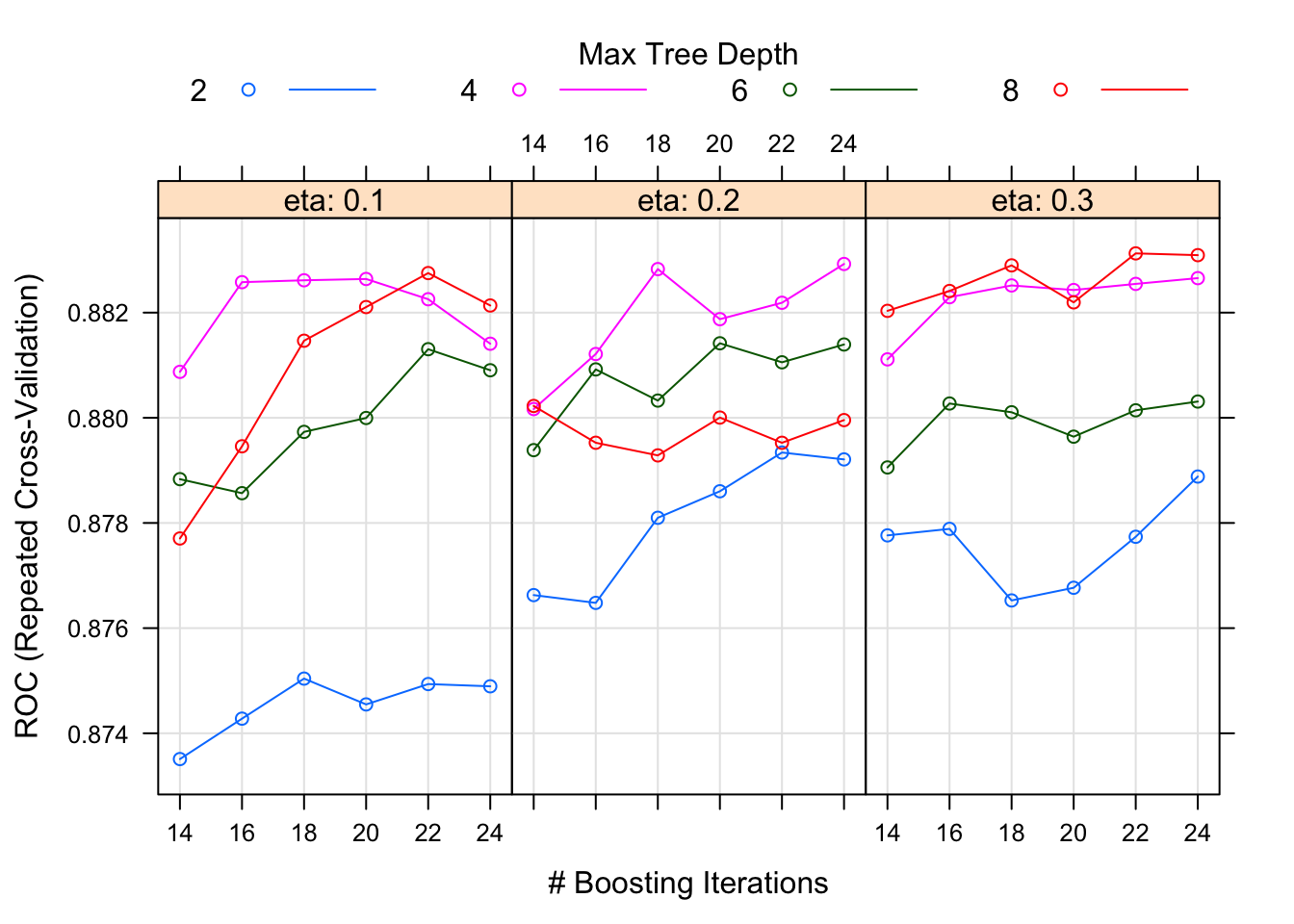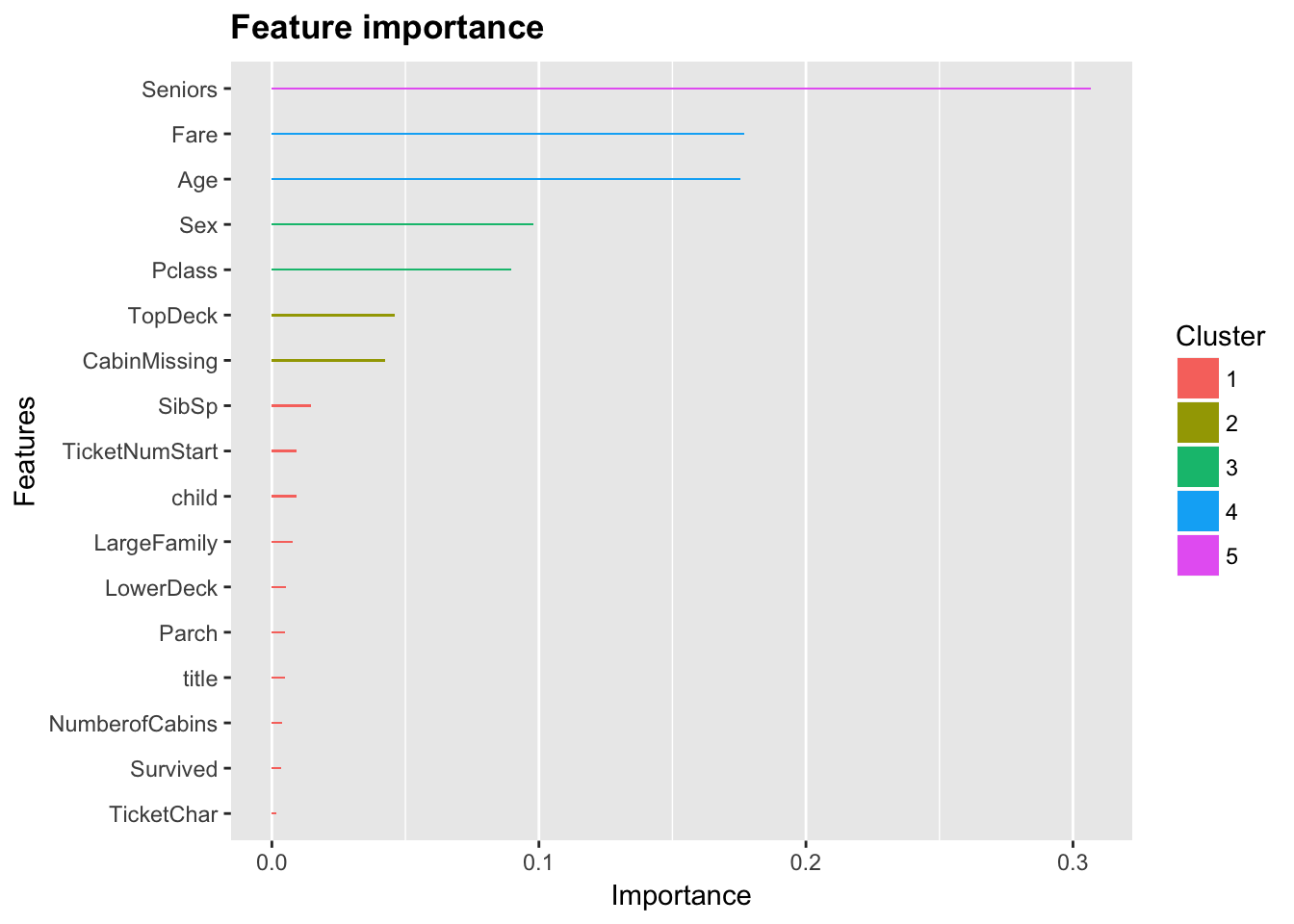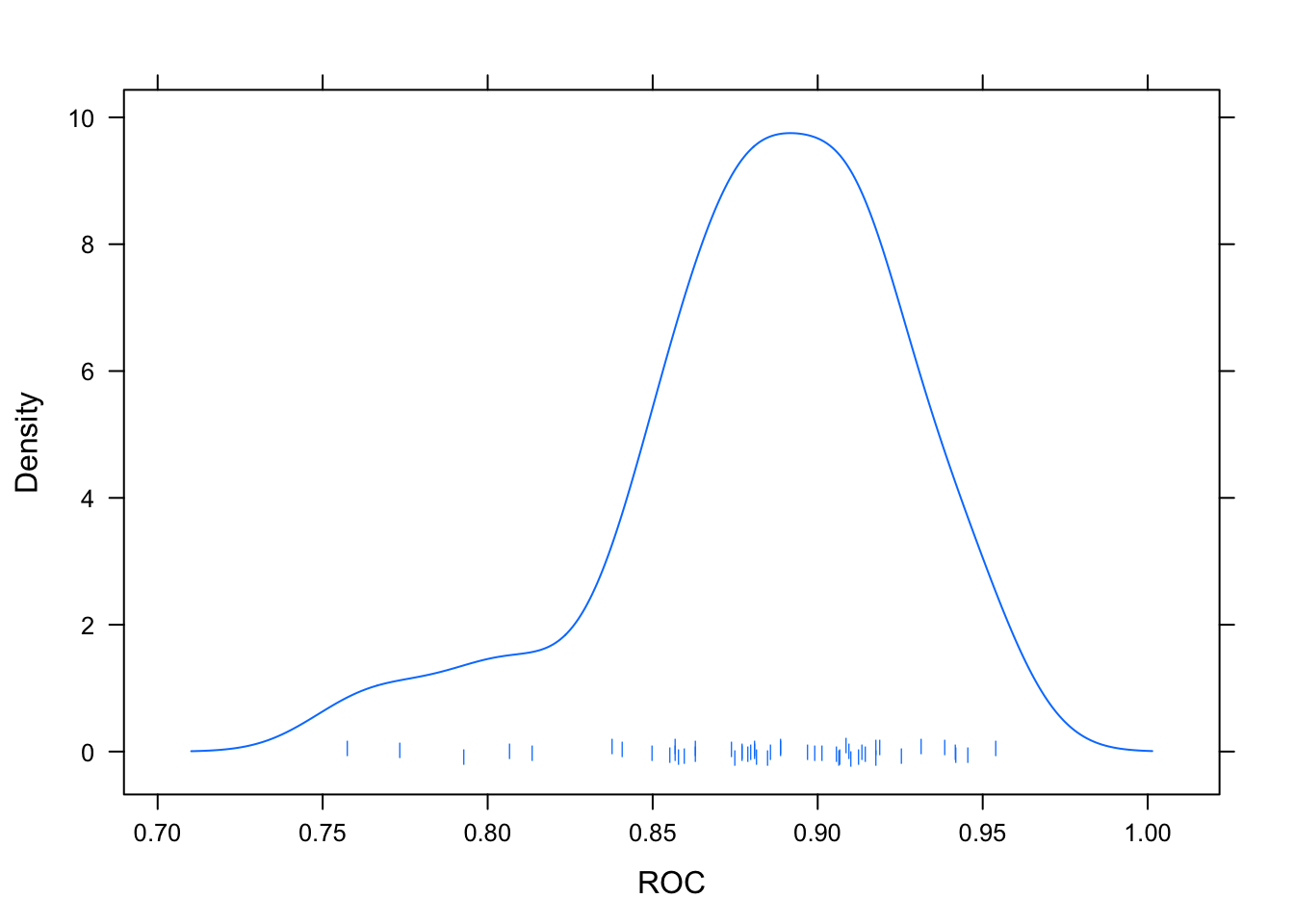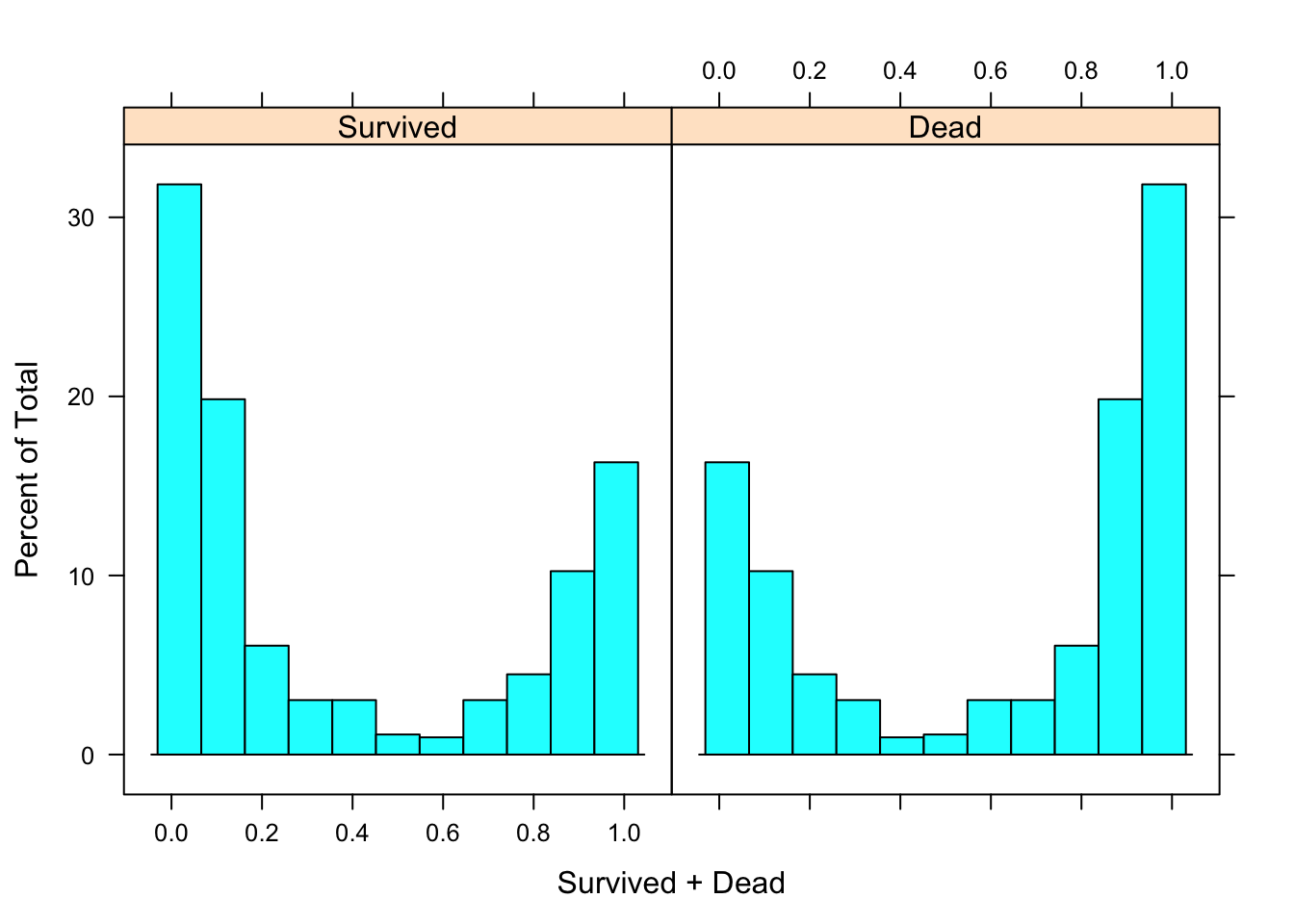## Elastinet

Moving on to a mixture model of ridge & lasso.

``````ctrl <- trainControl(method = "repeatedcv",
repeats = 5,
verboseIter = F,
classProbs = TRUE,
summaryFunction = twoClassSummary,
savePredictions = T
# sampling = 'down'
)
glmnetGrid <- expand.grid(.alpha = c(0,.2,.4,.6,.8,1),
.lambda = seq(10^-10,10^-1,0.02))
glmnetFit <- train(
Survived~.,
train.imp,
trControl=ctrl,
method='glmnet',
tuneGrid = glmnetGrid
)
save(glmnetFit,file = 'glmnetFit')``````

What we can see:

• The final values used for the model were alpha = 1 and lambda = 0.02. An alpha of 1 indicates this is a pure lasso model
• Like before, I don’t like is how it doesn’t seem to tail off at either end, i.e. ~20% of the data in either category is strongly misclassified.
• A quick look at the var importance plot does show that the derived variables of title, ticket char, and ticket number are important. Not bad for some quick feature engineering.
``glmnetFit``
``````## glmnet
##
## 625 samples
##  22 predictor
##   2 classes: 'Survived', 'Dead'
##
## No pre-processing
## Resampling: Cross-Validated (10 fold, repeated 5 times)
## Summary of sample sizes: 563, 562, 563, 563, 562, 563, ...
## Resampling results across tuning parameters:
##
##   alpha  lambda  ROC        Sens       Spec
##   0.0    1e-10   0.8717698  0.7500000  0.8727935
##   0.0    2e-02   0.8717698  0.7500000  0.8727935
##   0.0    4e-02   0.8719369  0.7441667  0.8774629
##   0.0    6e-02   0.8726847  0.7375000  0.8795412
##   0.0    8e-02   0.8727477  0.7308333  0.8826451
##   0.2    1e-10   0.8626538  0.7575000  0.8463023
##   0.2    2e-02   0.8739066  0.7591667  0.8712551
##   0.2    4e-02   0.8738065  0.7450000  0.8753711
##   0.2    6e-02   0.8736283  0.7308333  0.8795951
##   0.2    8e-02   0.8714555  0.7133333  0.8795816
##   0.4    1e-10   0.8616377  0.7550000  0.8463023
##   0.4    2e-02   0.8740992  0.7583333  0.8675978
##   0.4    4e-02   0.8733361  0.7216667  0.8748718
##   0.4    6e-02   0.8700475  0.7108333  0.8758974
##   0.4    8e-02   0.8636204  0.7016667  0.8779487
##   0.6    1e-10   0.8615314  0.7533333  0.8463023
##   0.6    2e-02   0.8741402  0.7525000  0.8696356
##   0.6    4e-02   0.8725290  0.7108333  0.8769366
##   0.6    6e-02   0.8632900  0.7025000  0.8758839
##   0.6    8e-02   0.8630294  0.7041667  0.8691363
##   0.8    1e-10   0.8615742  0.7533333  0.8463023
##   0.8    2e-02   0.8752567  0.7325000  0.8712146
##   0.8    4e-02   0.8691217  0.7125000  0.8790013
##   0.8    6e-02   0.8645069  0.7058333  0.8665452
##   0.8    8e-02   0.8645504  0.7050000  0.8406208
##   1.0    1e-10   0.8614454  0.7541667  0.8463023
##   1.0    2e-02   0.8758111  0.7241667  0.8753711
##   1.0    4e-02   0.8650436  0.7150000  0.8712011
##   1.0    6e-02   0.8633617  0.7066667  0.8456815
##   1.0    8e-02   0.8545662  0.7008333  0.8328340
##
## ROC was used to select the optimal model using the largest value.
## The final values used for the model were alpha = 1 and lambda = 0.02.``````
``````glmnPlot <- plot(glmnetFit,
plotType = "level",
cuts = 15,
scales = list(x = list(rot = 90, cex = .65)))
update(glmnPlot,
xlab = "Mixing Percentage\nRidge <---------> Lasso",
sub = "",
main = "Area Under the ROC Curve",
ylab = "Amount of Regularization")
densityplot(glmnetFit,pch='|')
plot(varImp(glmnetFit),15,main='Elastinet Model')
predict(glmnetFit,type = 'prob') -> train.glmnet.Probs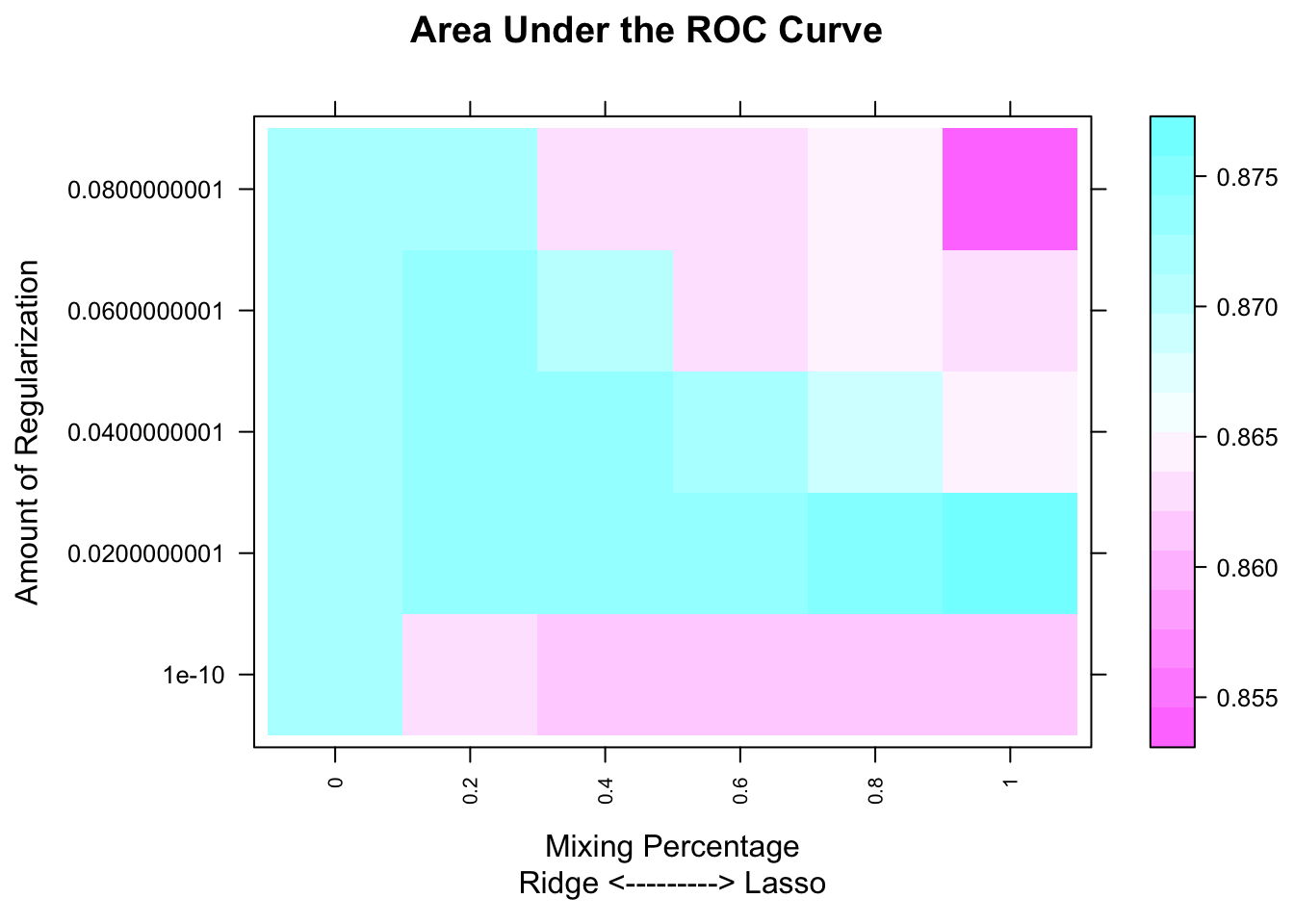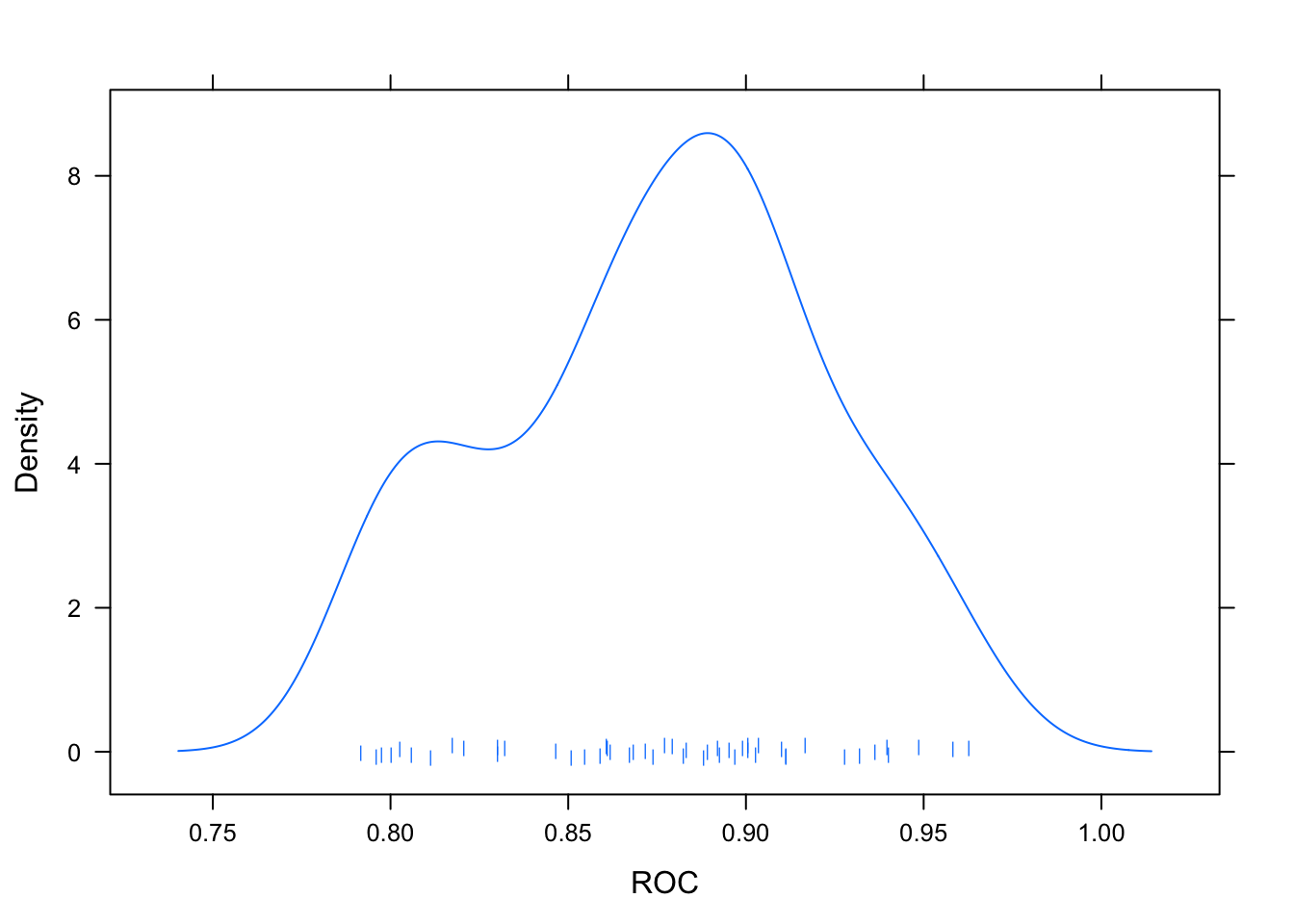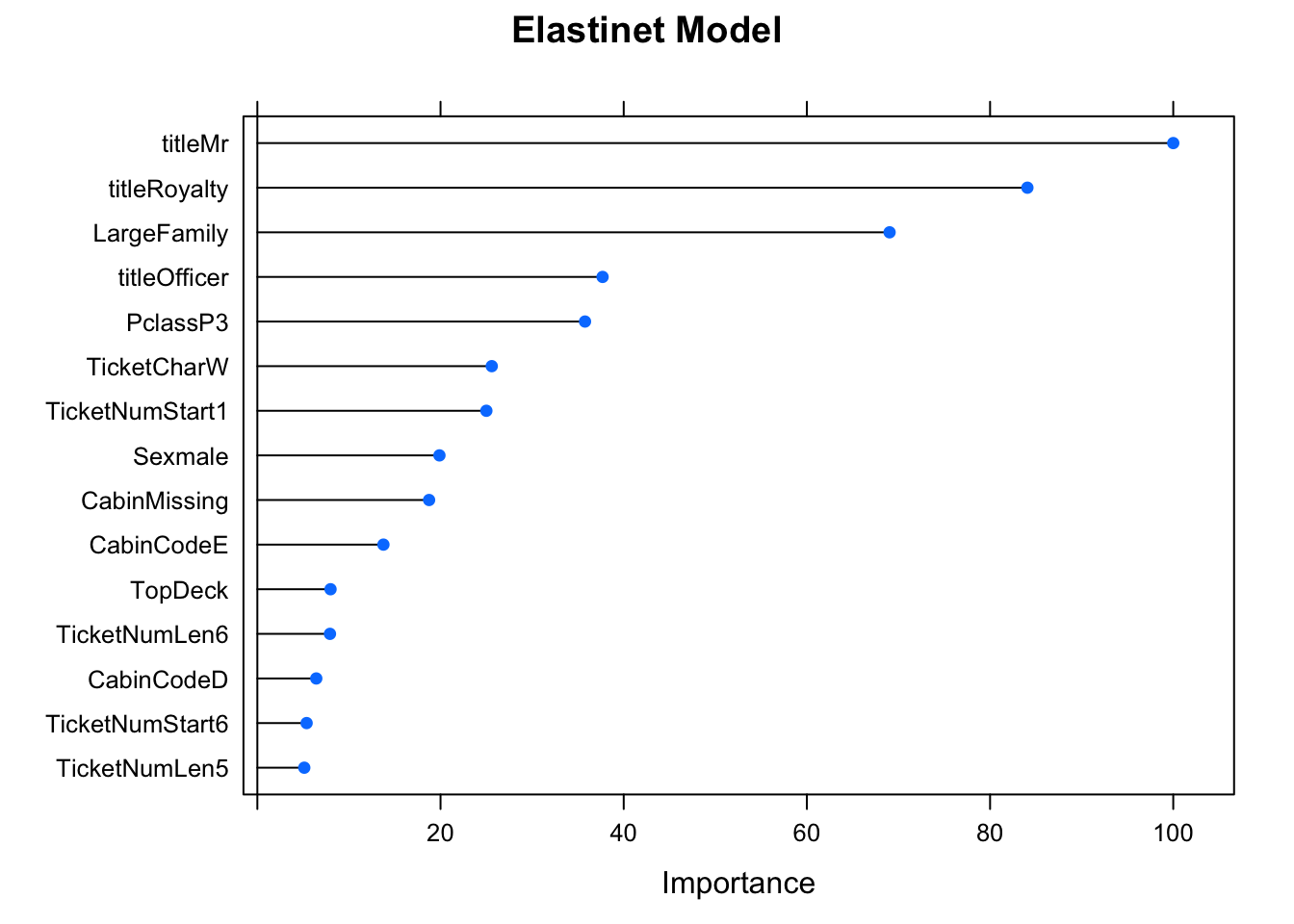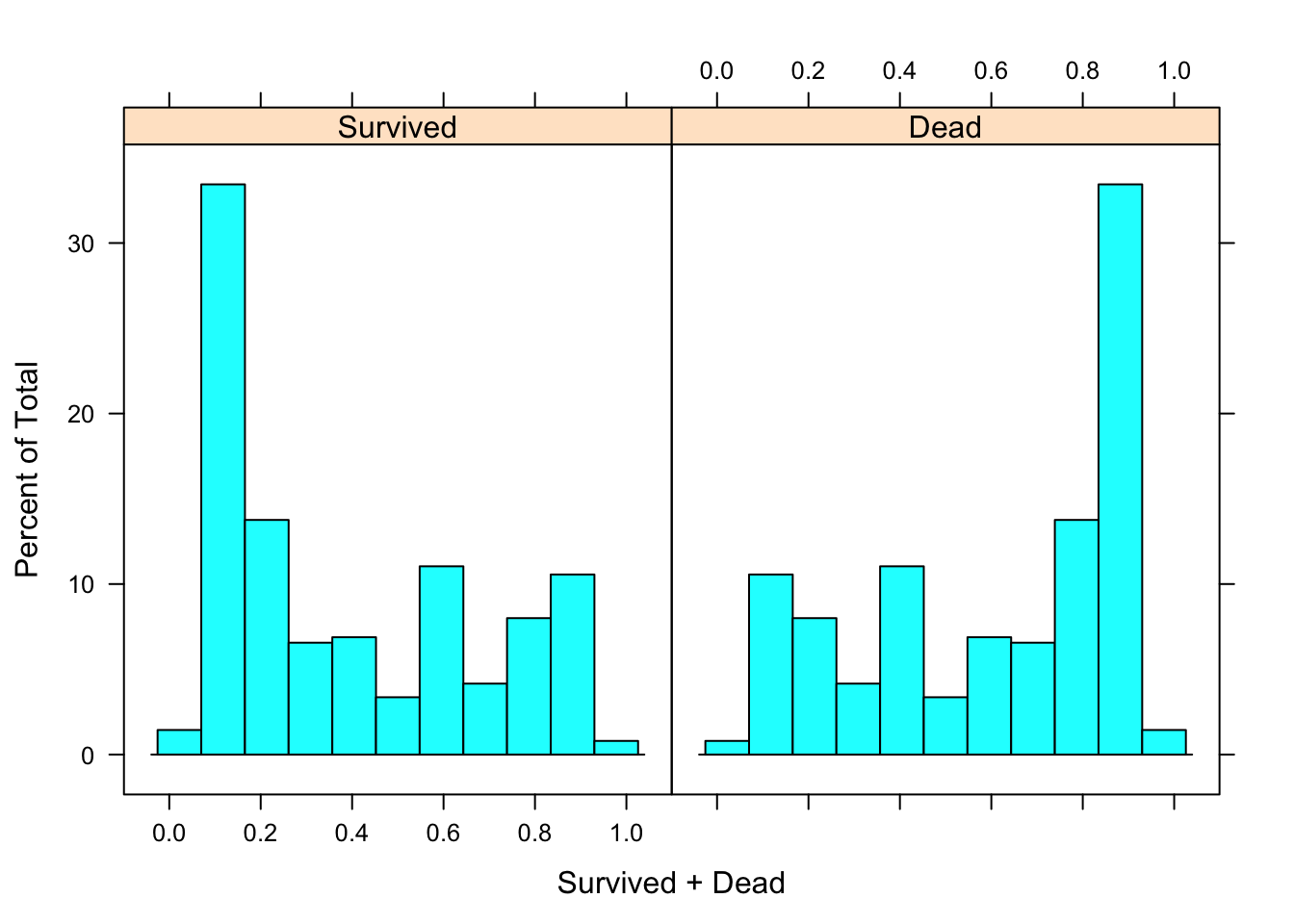## k-NN

`kNN` is expected to be the worst of the lot, and it doesn’t prove us wrong.

``````ctrl <- trainControl(method = "repeatedcv",
repeats = 5,
verboseIter = F,
classProbs = TRUE,
summaryFunction = twoClassSummary,
savePredictions = T
# sampling = 'down'
)
knnGrid <- expand.grid(k=seq(3,23,2))
knnFit <- train(
Survived~.,
train.imp,
method = 'knn',
trControl = ctrl,
tuneGrid = knnGrid
)``````
``````## Warning in train.default(x, y, weights = w, ...): The metric "Accuracy" was
## not in the result set. ROC will be used instead.``````
``save(knnFit,file = 'knnFit')``

The probability value histograms show how large the overlap is between the two classes. Clearly a poor predictor model.

``knnFit``
``````## k-Nearest Neighbors
##
## 625 samples
##  22 predictor
##   2 classes: 'Survived', 'Dead'
##
## No pre-processing
## Resampling: Cross-Validated (10 fold, repeated 5 times)
## Summary of sample sizes: 563, 562, 563, 562, 562, 563, ...
## Resampling results across tuning parameters:
##
##   k   ROC        Sens       Spec
##    3  0.7587697  0.5908333  0.8027530
##    5  0.7597366  0.5800000  0.8125236
##    7  0.7614229  0.5816667  0.8083941
##    9  0.7668756  0.5975000  0.8047233
##   11  0.7693668  0.5808333  0.8129825
##   13  0.7703925  0.5550000  0.8311876
##   15  0.7754467  0.5416667  0.8239406
##   17  0.7777497  0.5491667  0.8297166
##   19  0.7746016  0.5408333  0.8380027
##   21  0.7708986  0.5516667  0.8452227
##   23  0.7661907  0.5391667  0.8488529
##
## ROC was used to select the optimal model using the largest value.
## The final value used for the model was k = 17.``````
``````plot(knnFit)
densityplot(knnFit,pch='|')
predict(knnFit,type = 'prob') -> train.Probs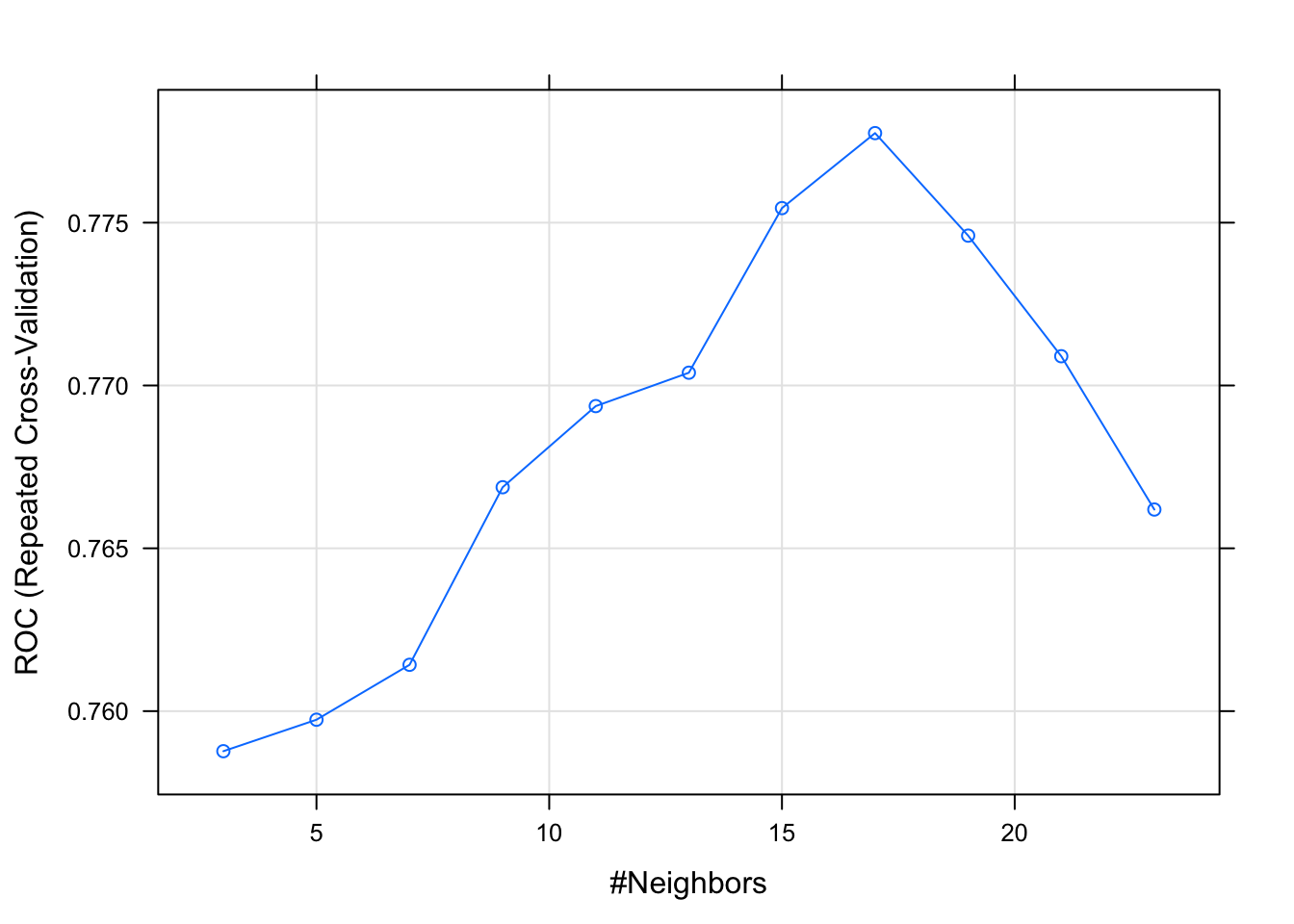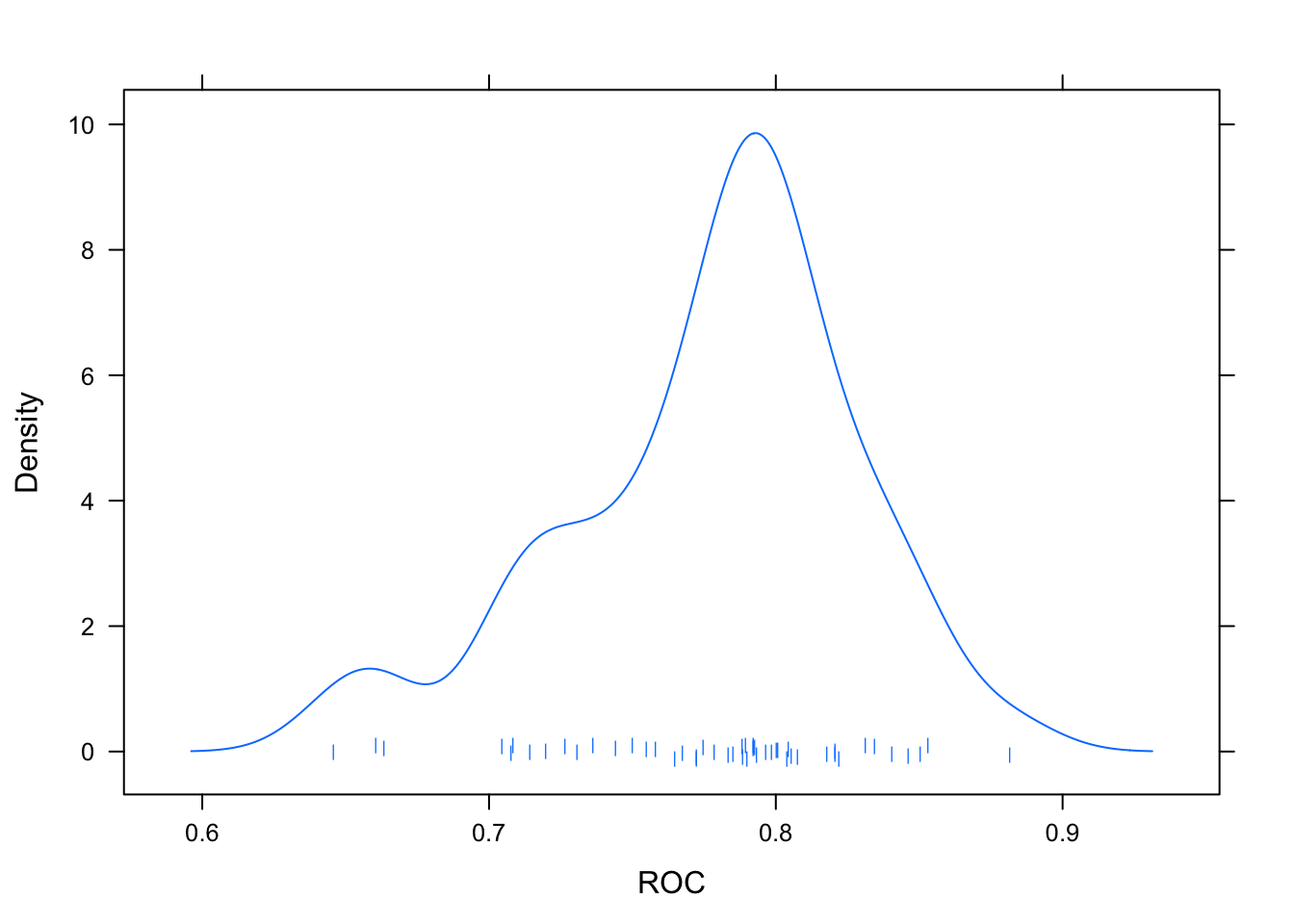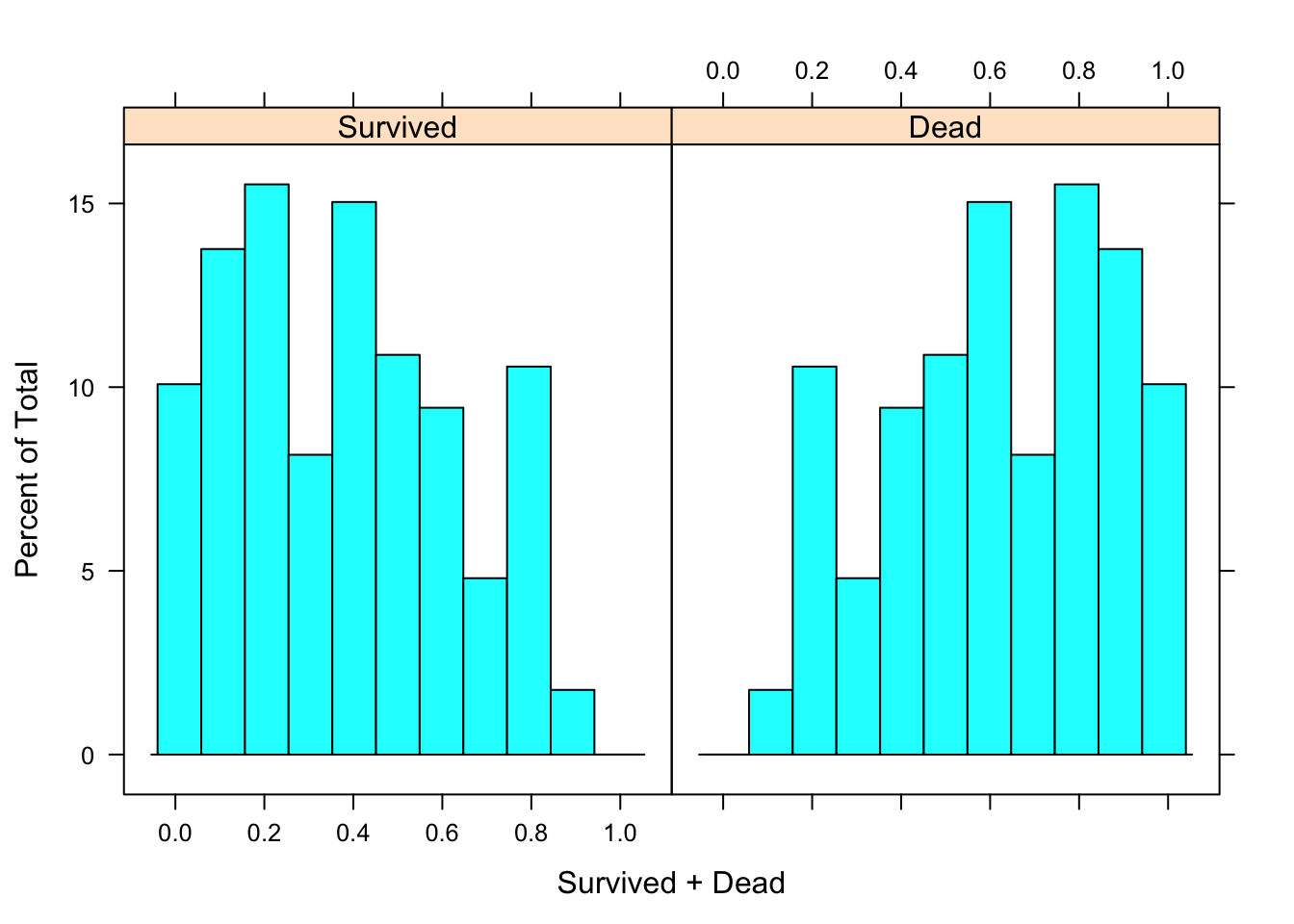## SVM

``````ctrl <- trainControl(method = "repeatedcv",
repeats = 5,
verboseIter = F,
classProbs = TRUE,
summaryFunction = twoClassSummary,
savePredictions = T
# sampling = 'down'
)
svmFit <- train(
Survived~.,
train.imp,
trControl = ctrl,
tuneGrid = expand.grid(C=c(0.05,0.1,0.2,0.3), sigma=c(0.001,0.005,0.01,0.015))
)
save(svmFit,file = 'svmFit')``````
• The SVM shows moderate performance, but notice many wrongs it gets confidently. Large peaks (~20%) in the probability histograms indicate that the predictions this model gets wrong, it gets wrong very confidently.
``svmFit``
``````## Support Vector Machines with Radial Basis Function Kernel
##
## 625 samples
##  22 predictor
##   2 classes: 'Survived', 'Dead'
##
## No pre-processing
## Resampling: Cross-Validated (10 fold, repeated 5 times)
## Summary of sample sizes: 562, 562, 562, 563, 562, 563, ...
## Resampling results across tuning parameters:
##
##   C     sigma  ROC        Sens       Spec
##   0.05  0.001  0.8112719  0.7275000  0.7859379
##   0.05  0.005  0.8197903  0.7483333  0.7740081
##   0.05  0.010  0.8271795  0.7541667  0.7766667
##   0.05  0.015  0.8308153  0.7575000  0.7854926
##   0.10  0.001  0.8129605  0.7250000  0.7900675
##   0.10  0.005  0.8197886  0.7483333  0.7734953
##   0.10  0.010  0.8288906  0.7366667  0.7927260
##   0.10  0.015  0.8316341  0.7466667  0.7922267
##   0.20  0.001  0.8167904  0.7233333  0.7931579
##   0.20  0.005  0.8407428  0.6858333  0.8363698
##   0.20  0.010  0.8424190  0.6741667  0.8562618
##   0.20  0.015  0.8422610  0.6808333  0.8505668
##   0.30  0.001  0.8190896  0.7275000  0.7962483
##   0.30  0.005  0.8438647  0.6325000  0.8748178
##   0.30  0.010  0.8467133  0.6333333  0.8837517
##   0.30  0.015  0.8447093  0.6633333  0.8666937
##
## ROC was used to select the optimal model using the largest value.
## The final values used for the model were sigma = 0.01 and C = 0.3.``````
``````plot(svmFit)
densityplot(svmFit,pch='|')
predict(svmFit,type = 'prob') -> train.Probs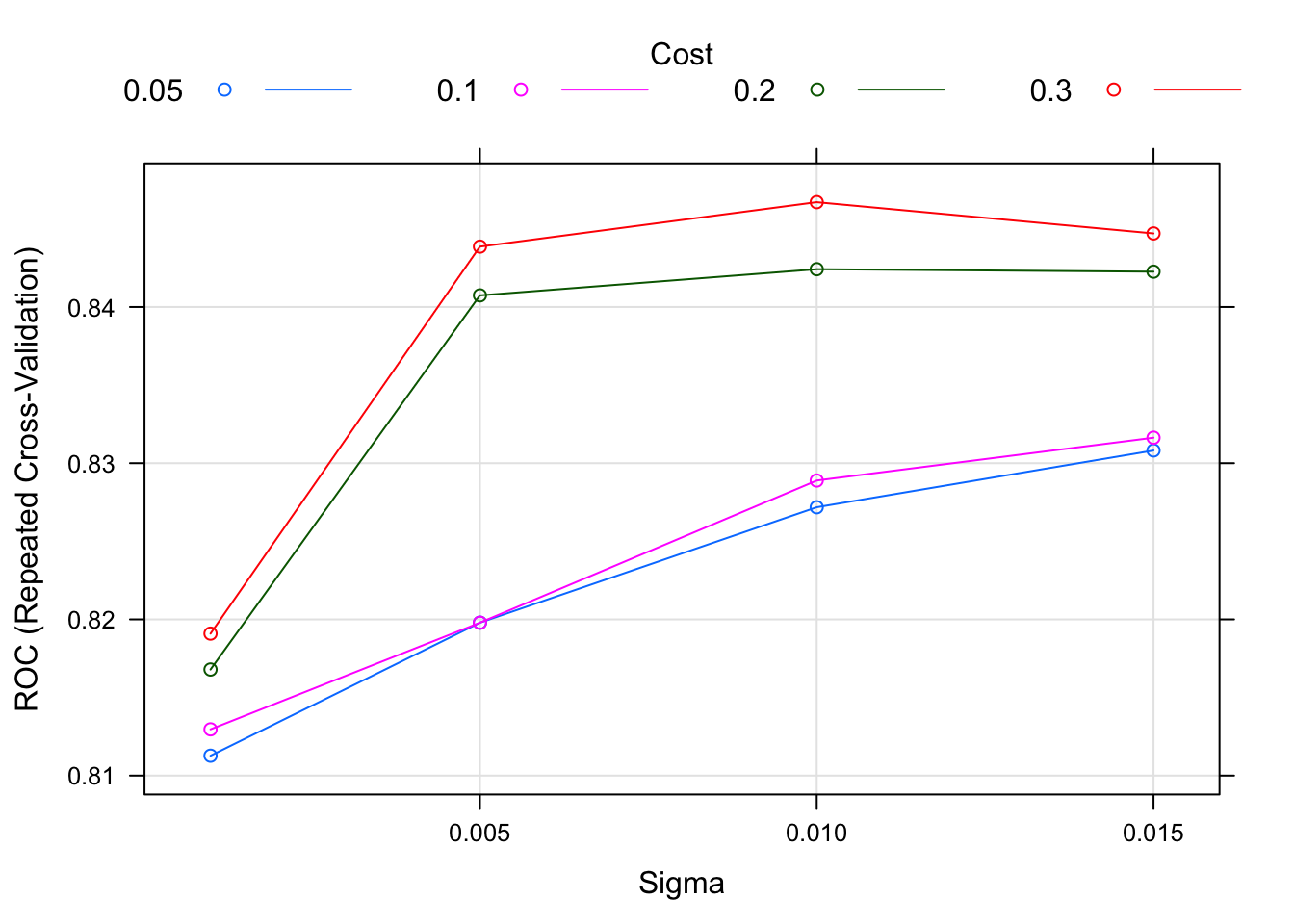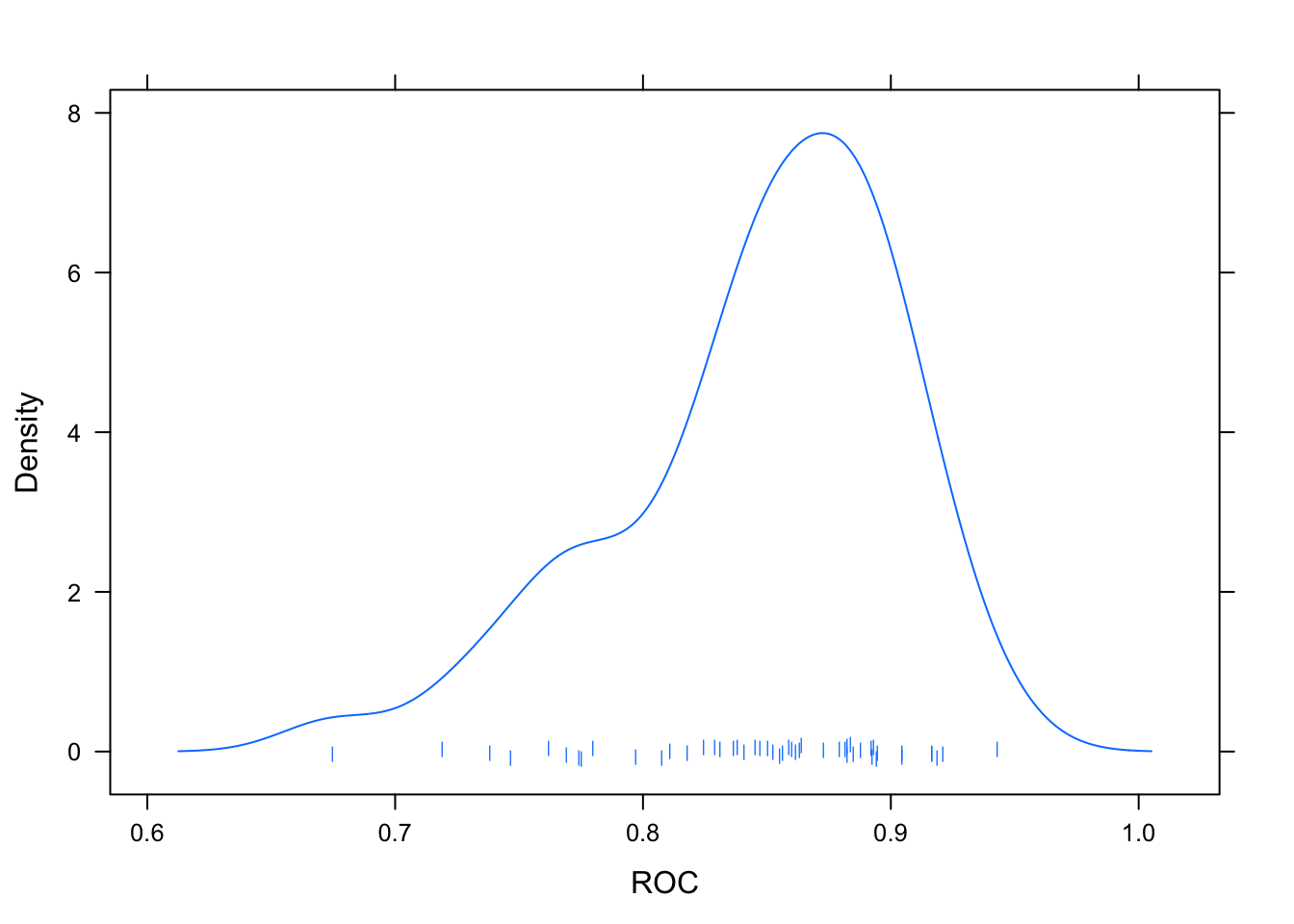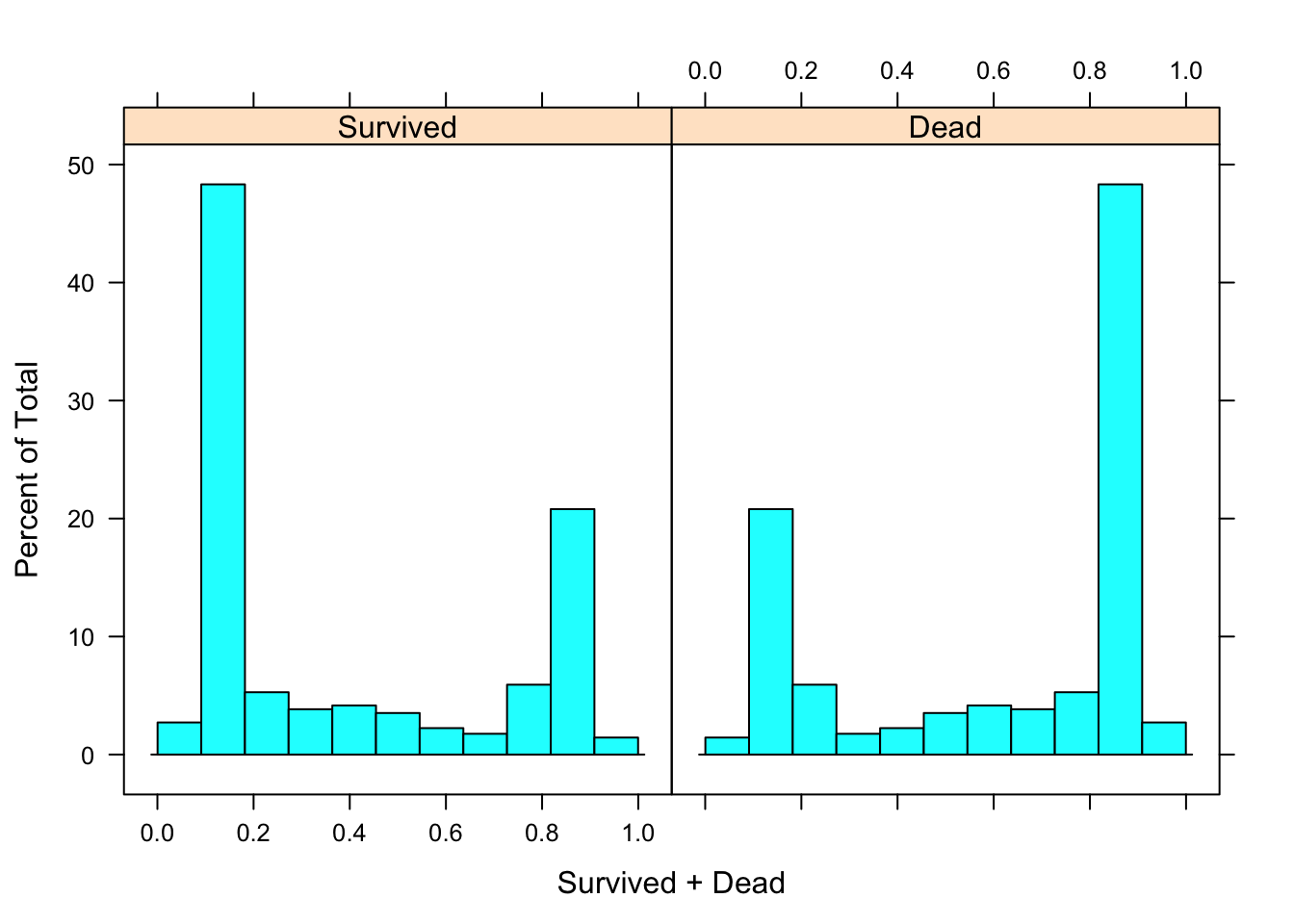## C5.0

``````ctrl <- trainControl(method = "repeatedcv",
repeats = 5,
verboseIter = F,
classProbs = TRUE,
summaryFunction = twoClassSummary,
savePredictions = T
)
c5Fit <- train(
Survived~.,
train.imp,
method = 'C5.0',
trControl = ctrl,
control = C50::C5.0Control(earlyStopping = FALSE)
)``````
``````## Warning in train.default(x, y, weights = w, ...): The metric "Accuracy" was
## not in the result set. ROC will be used instead.``````
``save(c5Fit,file = 'c5Fit')``
• For the C5.0 model, I’ve kept the grid search space the default offered by `caret`.
• We still see the ‘U’ shape on the probability distributions, although more spread out than what we saw in the SVM model.
``c5Fit``
``````## C5.0
##
## 625 samples
##  22 predictor
##   2 classes: 'Survived', 'Dead'
##
## No pre-processing
## Resampling: Cross-Validated (10 fold, repeated 5 times)
## Summary of sample sizes: 562, 562, 563, 563, 562, 563, ...
## Resampling results across tuning parameters:
##
##   model  winnow  trials  ROC        Sens       Spec
##   rules  FALSE    1      0.8350037  0.7266667  0.8888664
##   rules  FALSE   10      0.8794467  0.7575000  0.8810256
##   rules  FALSE   20      0.8811004  0.7558333  0.8893117
##   rules   TRUE    1      0.8192578  0.7175000  0.8768556
##   rules   TRUE   10      0.8750616  0.7350000  0.8778947
##   rules   TRUE   20      0.8768250  0.7458333  0.8877868
##   tree   FALSE    1      0.8595808  0.7141667  0.8888529
##   tree   FALSE   10      0.8739195  0.7383333  0.8899055
##   tree   FALSE   20      0.8772911  0.7408333  0.8872740
##   tree    TRUE    1      0.8496924  0.7191667  0.8721457
##   tree    TRUE   10      0.8713200  0.7383333  0.8851687
##   tree    TRUE   20      0.8765562  0.7508333  0.8815115
##
## ROC was used to select the optimal model using the largest value.
## The final values used for the model were trials = 20, model = rules
##  and winnow = FALSE.``````
``````plot(c5Fit)
densityplot(c5Fit,pch='|')
predict(c5Fit,type = 'prob') -> train.Probs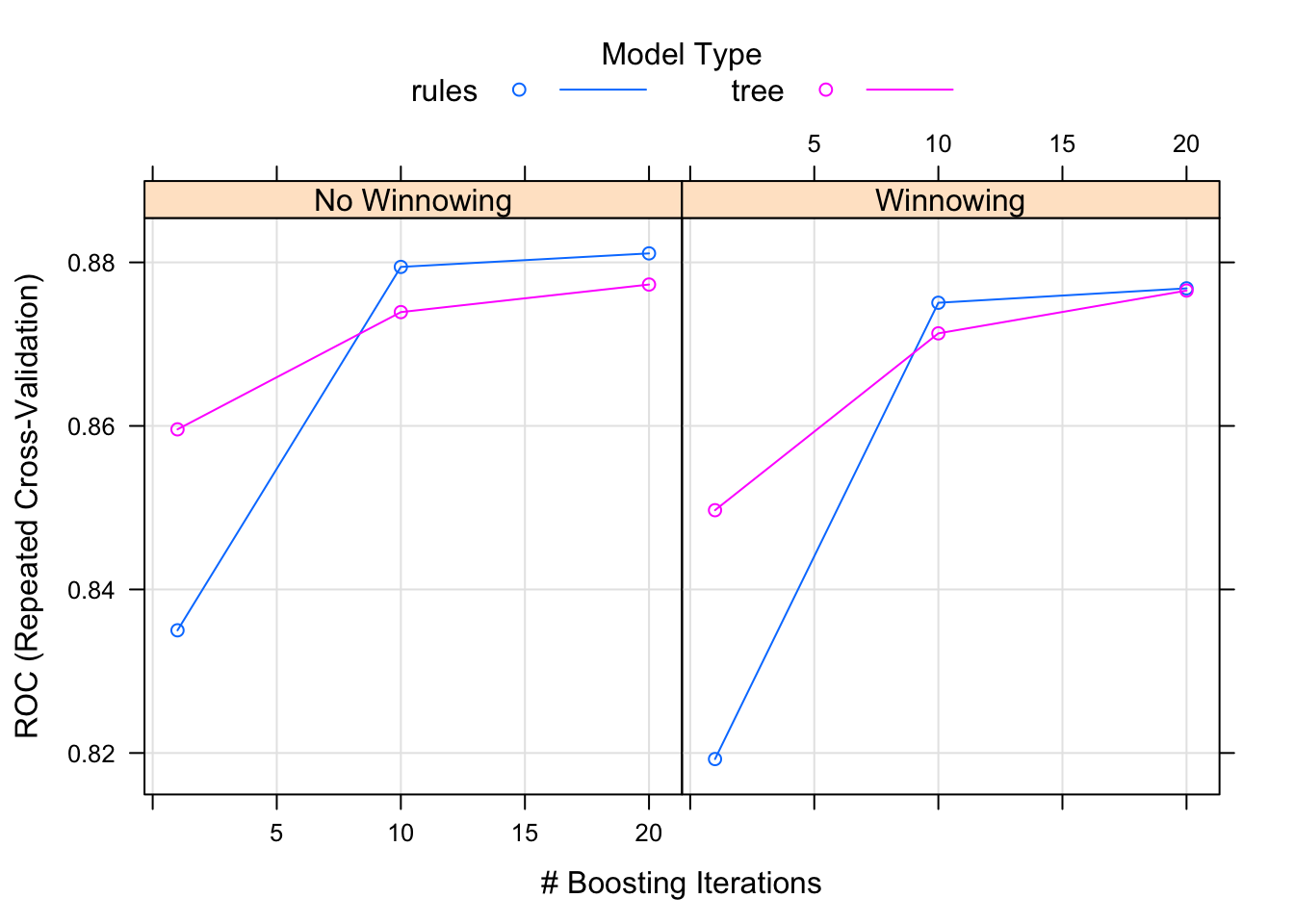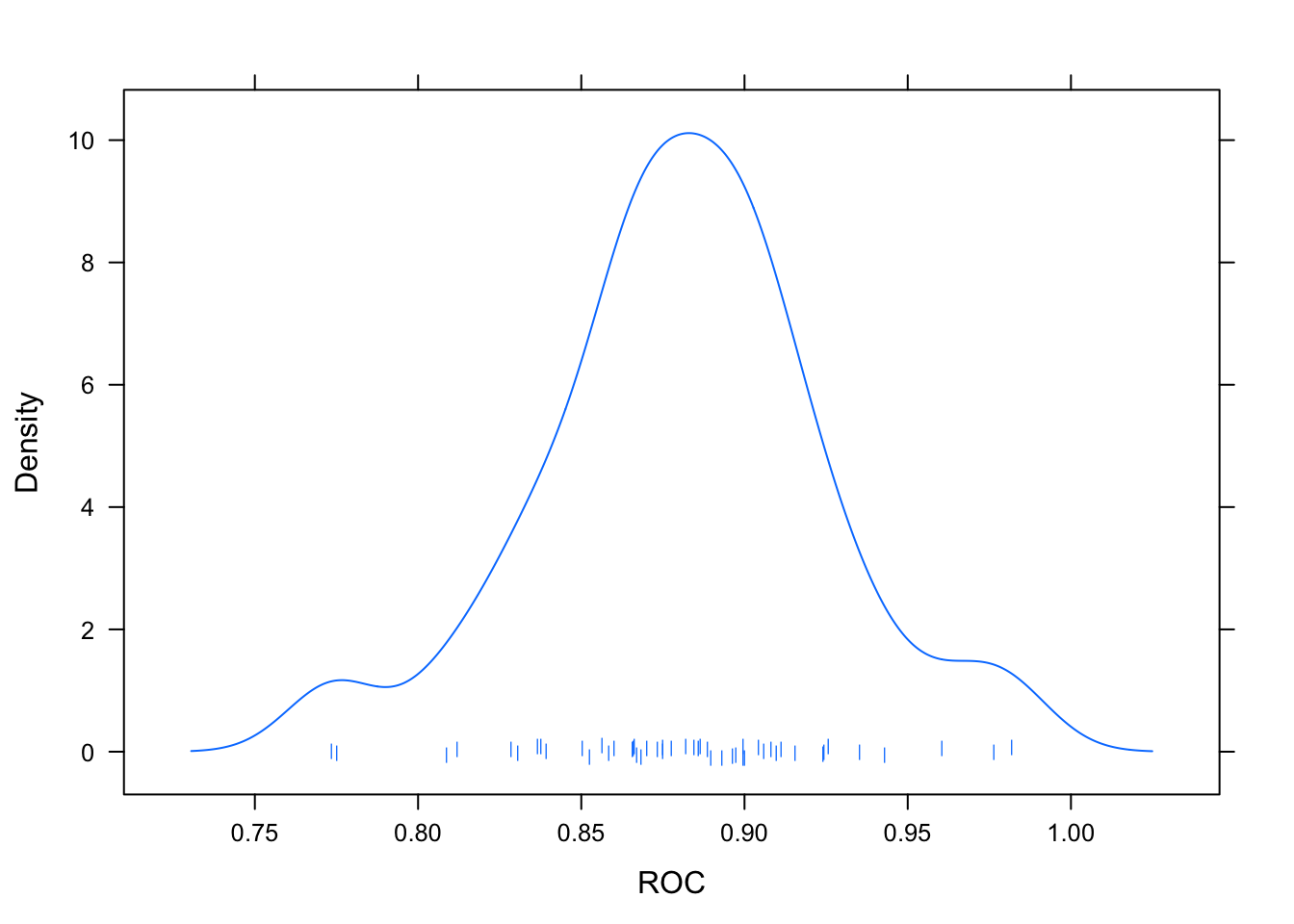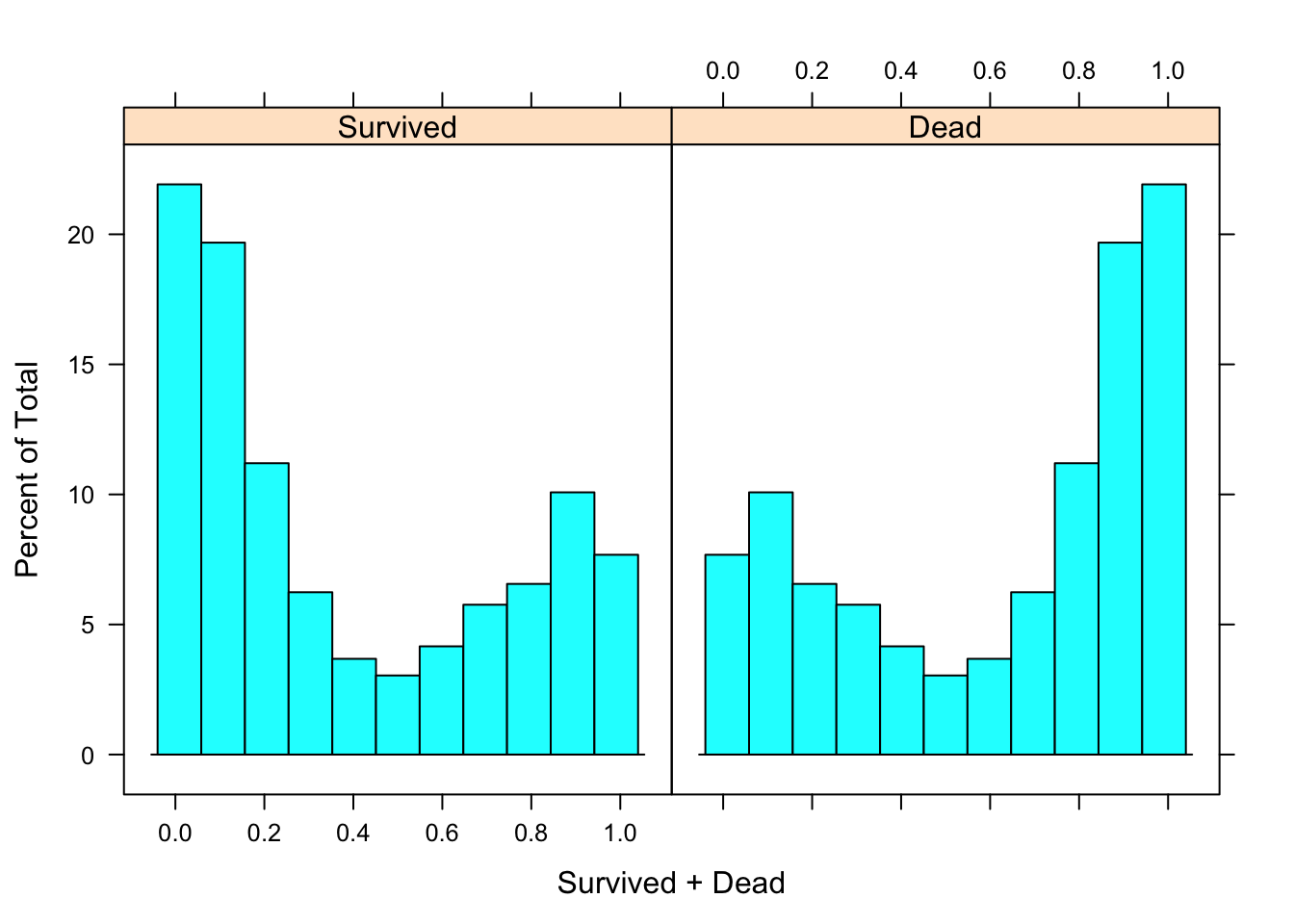## Averaged Neural Networks

``````ctrl <- trainControl(method = "repeatedcv",
repeats = 5,
classProbs = TRUE,
summaryFunction = twoClassSummary,
savePredictions = T
)
avNNetgrid <- expand.grid(size=c(2,4,6,8),decay=c(0.1,0.2),bag=c(T,F))
avNnetFit <- train(Survived~.,
train.imp,
method = "avNNet",
trControl = ctrl,
tuneGrid = avNNetgrid,
repeats = 15,
trace = FALSE)
save(avNnetFit,file = 'avNnetFit')``````

Averaged neural networks are something I picked up in the 2018 RStudio conference. It fits multiple neural networks to a problem and returns the average value of all the nnets together. Easy to use, pretty spiffy.

There are three tunable parameters here - # of hidden nodes, bagging and weight decay. Upon playing with them a bit, I settled on what you see below. Perhaps increasing the # of hidden units would have made more of a difference, but I’m happy with this so far.

``avNnetFit``
``````## Model Averaged Neural Network
##
## 625 samples
##  22 predictor
##   2 classes: 'Survived', 'Dead'
##
## No pre-processing
## Resampling: Cross-Validated (10 fold, repeated 5 times)
## Summary of sample sizes: 562, 563, 563, 562, 562, 562, ...
## Resampling results across tuning parameters:
##
##   size  decay  bag    ROC        Sens       Spec
##   2     0.1    FALSE  0.8741481  0.7283333  0.8956410
##   2     0.1     TRUE  0.8745440  0.7266667  0.8935088
##   2     0.2    FALSE  0.8747430  0.7283333  0.9002834
##   2     0.2     TRUE  0.8760897  0.7300000  0.8924426
##   4     0.1    FALSE  0.8770547  0.7458333  0.8909582
##   4     0.1     TRUE  0.8781877  0.7383333  0.8898516
##   4     0.2    FALSE  0.8776175  0.7383333  0.8956005
##   4     0.2     TRUE  0.8759807  0.7333333  0.8923887
##   6     0.1    FALSE  0.8765132  0.7400000  0.8898785
##   6     0.1     TRUE  0.8779892  0.7450000  0.8857490
##   6     0.2    FALSE  0.8777845  0.7391667  0.8934548
##   6     0.2     TRUE  0.8765373  0.7391667  0.8929150
##   8     0.1    FALSE  0.8754892  0.7416667  0.8851957
##   8     0.1     TRUE  0.8747813  0.7391667  0.8847233
##   8     0.2    FALSE  0.8773043  0.7366667  0.8945614
##   8     0.2     TRUE  0.8783103  0.7350000  0.8935358
##
## ROC was used to select the optimal model using the largest value.
## The final values used for the model were size = 8, decay = 0.2 and bag
##  = TRUE.``````
``````plot(avNnetFit)
densityplot(avNnetFit,pch='|')
predict(avNnetFit,type = 'prob') -> train.Probs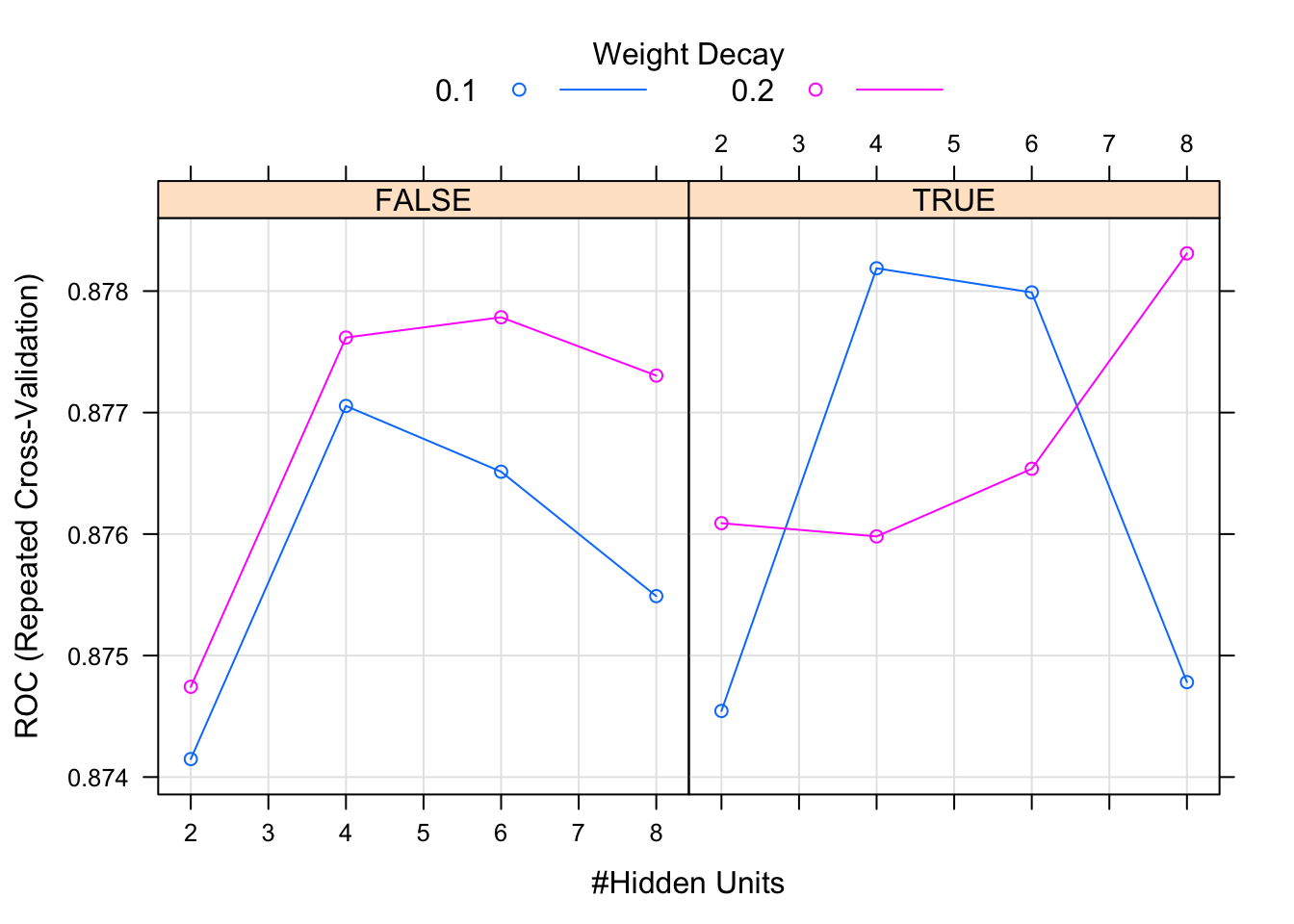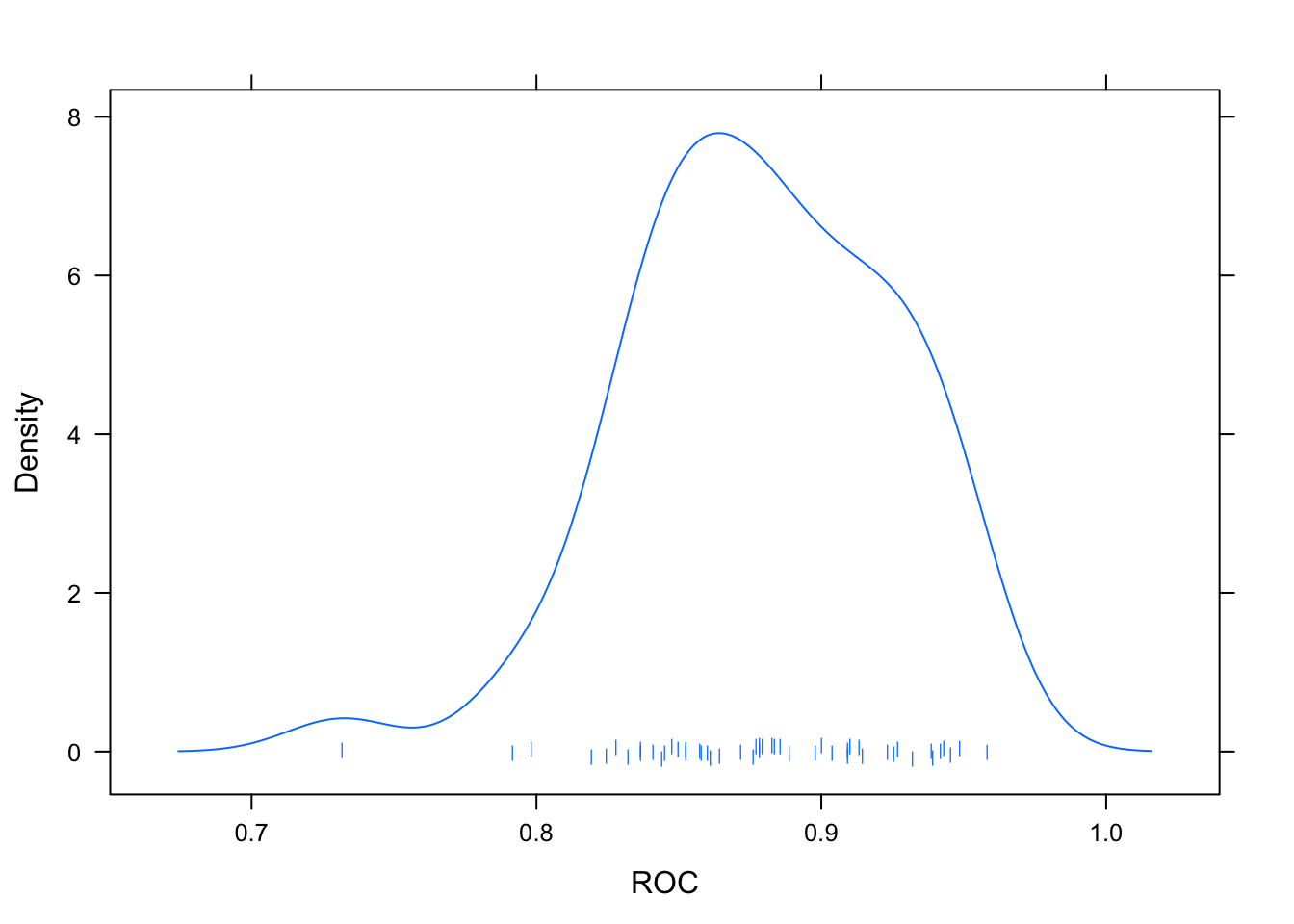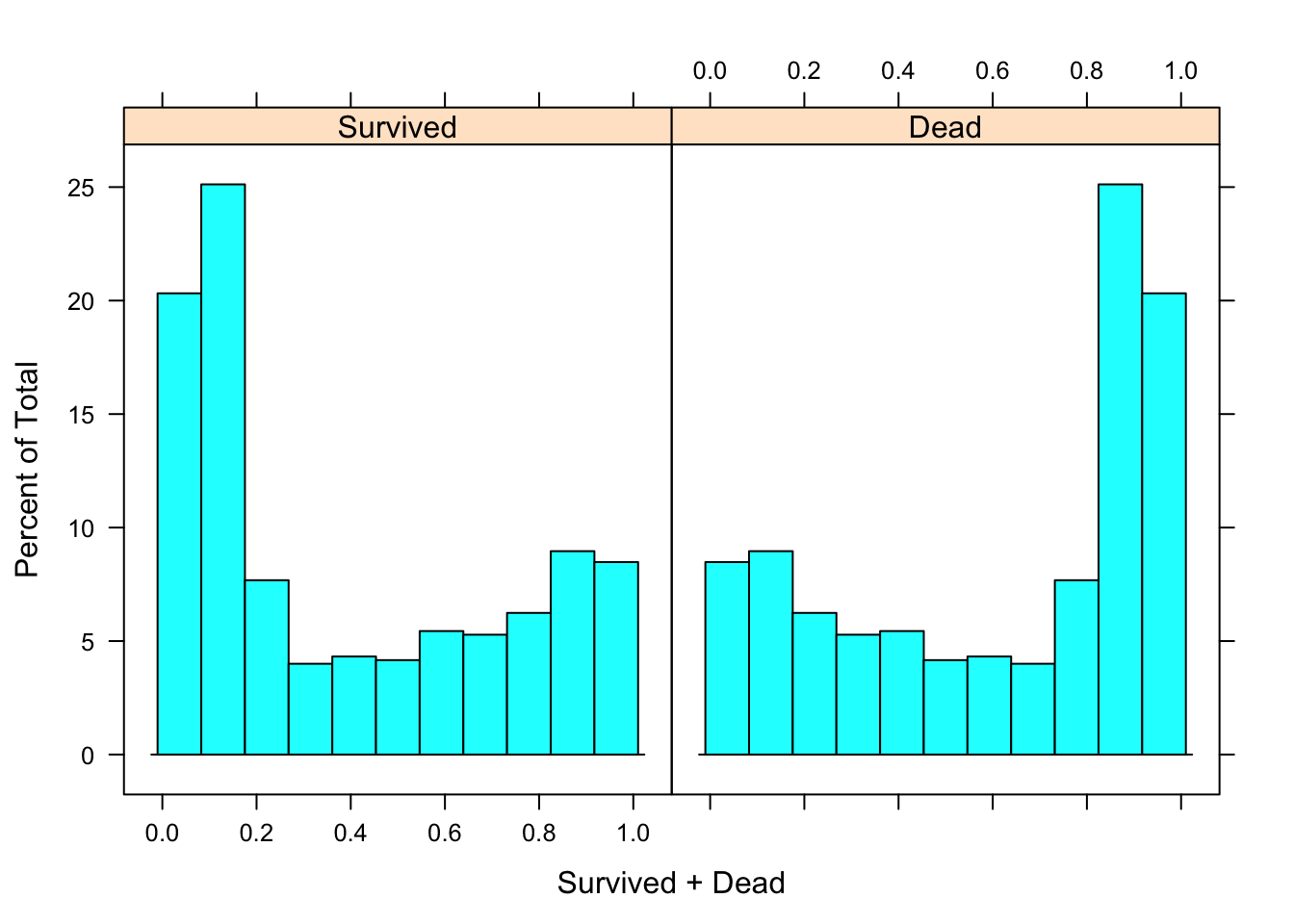## Conditional Inference Random Forests

``````ctrl <- trainControl(method = "repeatedcv",
repeats = 5,
verboseIter = F,
classProbs = TRUE,
summaryFunction = twoClassSummary,
savePredictions = T
)
ciRFgrid <- expand.grid(mtry = c(20,25,30,35,40))
ciRFFit <- train(Survived~.,
train.imp,
method = "cforest",
trControl = ctrl,
tuneGrid = ciRFgrid
) ``````
``````## Warning in train.default(x, y, weights = w, ...): The metric "Accuracy" was
## not in the result set. ROC will be used instead.``````
``save(ciRFFit,file = 'ciRFFit')``

This is another technology I picked up from converstaions at RStudio conference. I believe it’s referenced in the APM book as well. Some information about them is here. To be honest, I haven’t spent any time understanding exactly how these work, so to me they are black box(y) at the moment.

``ciRFFit``
``````## Conditional Inference Random Forest
##
## 625 samples
##  22 predictor
##   2 classes: 'Survived', 'Dead'
##
## No pre-processing
## Resampling: Cross-Validated (10 fold, repeated 5 times)
## Summary of sample sizes: 563, 563, 562, 563, 563, 562, ...
## Resampling results across tuning parameters:
##
##   mtry  ROC        Sens       Spec
##   20    0.8764103  0.7141667  0.8784211
##   25    0.8784511  0.7275000  0.8805533
##   30    0.8801816  0.7375000  0.8815924
##   35    0.8804375  0.7208333  0.8826451
##   40    0.8810473  0.7300000  0.8862753
##
## ROC was used to select the optimal model using the largest value.
## The final value used for the model was mtry = 40.``````
``````plot(ciRFFit)
densityplot(ciRFFit,pch='|')
predict(ciRFFit,type = 'prob') -> train.Probs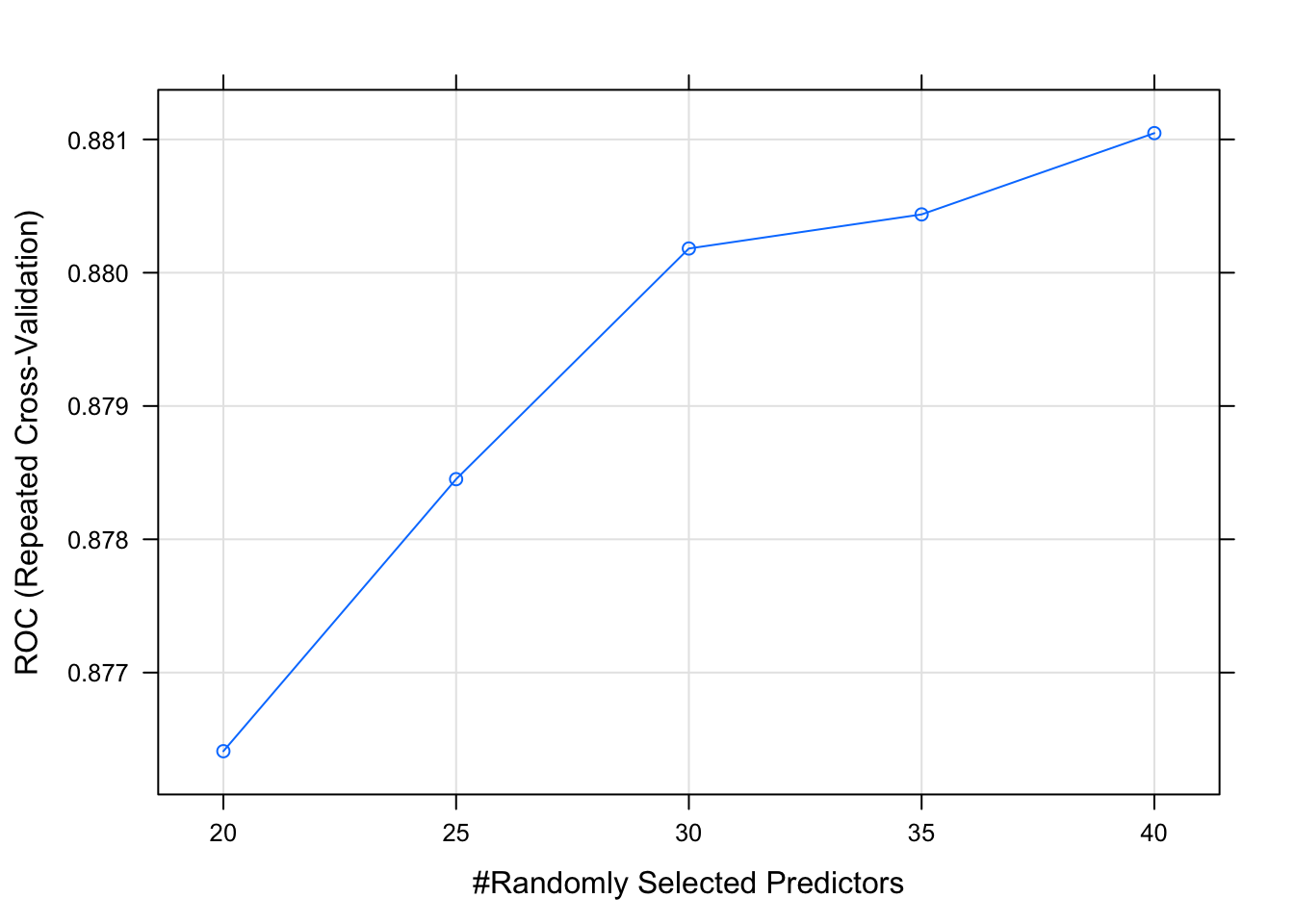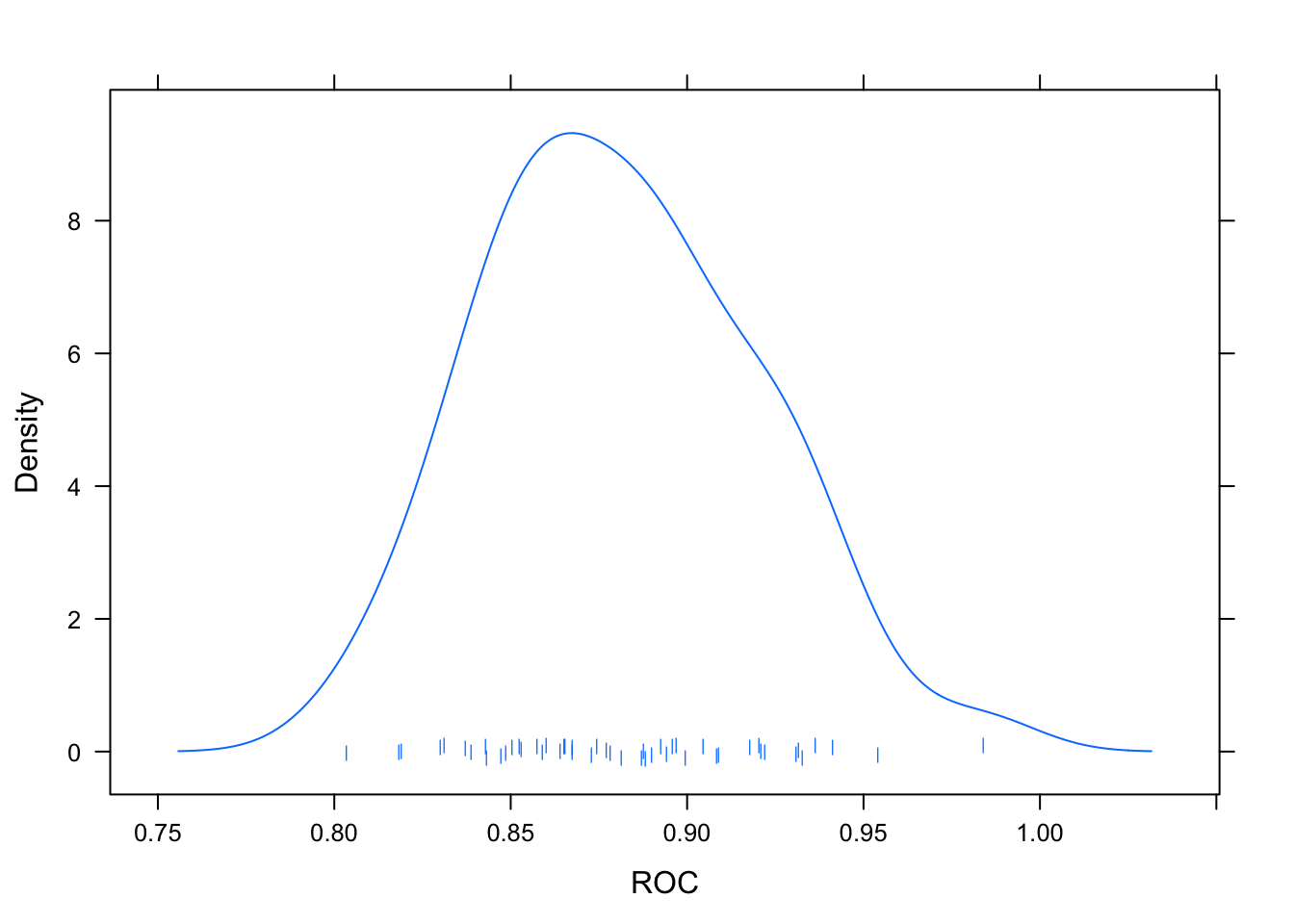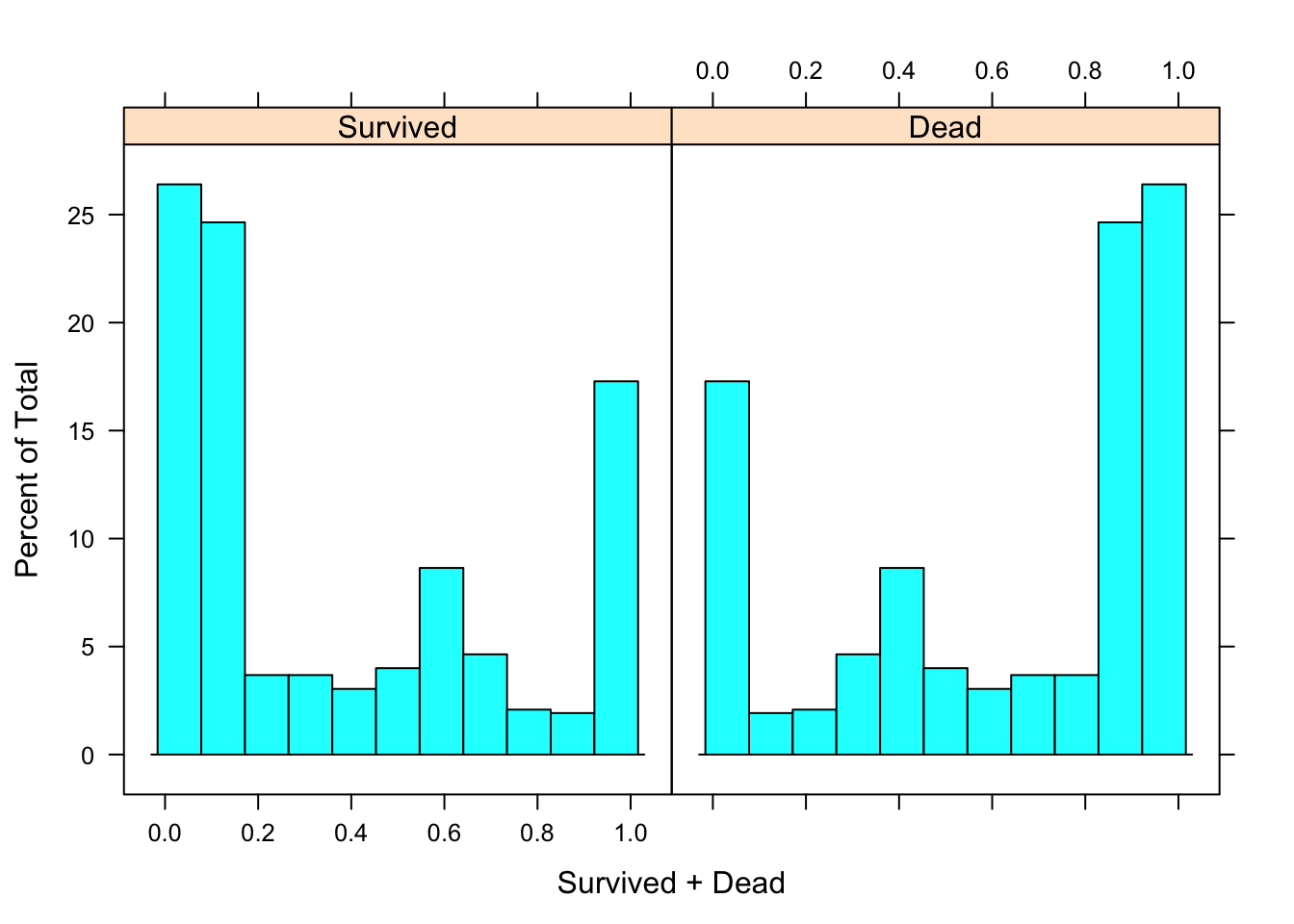# Compare models

Now the fun part - pitting these models against each other to figure out who’s the strongest. The beauty of using `caret` to analyze all the models with the same resampling strategy is that it allows one to use the `resamples()` function to quickly extract all the information in the fits and plot out key performance parameters like ROC, sensitivity and specificity.

``````re <-
resamples(x = list(
xgb = xgbFit,
knn = knnFit,
elastinet = glmnetFit,
C50 = c5Fit,
svm = svmFit,
avgNNet = avNnetFit,
ciRF = ciRFFit
))``````

This plot shows the point estimates (mean and CI of mean) of all three metrics, as evaluated on each repeated cross-validation on all models run per model-type. All the top 4 models are really alike in AUC ROC, with xgb and C50 taking a slight lead in sensitivity.

``dotplot(re)``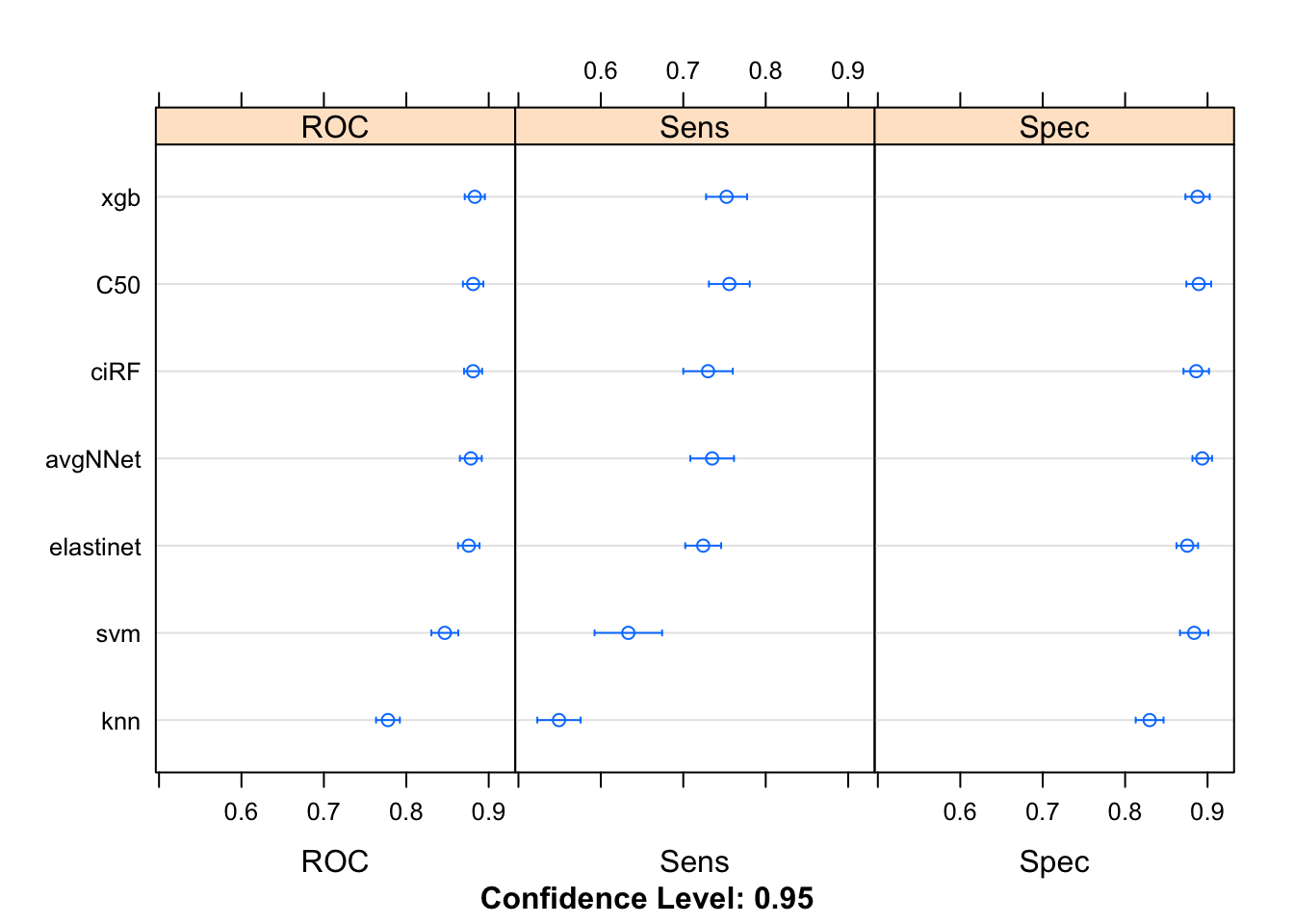Another way to visualize the data is to plot all the results of each model-type for all it’s cross-validated results on a boxplot. This shows us how much variation we might expect from the model for a true unknown test set. Here, elastinet seems to have the tightest IQR for AUC ROC as well as the tightest IQR for sensitivity. It also shows no outliers.

``bwplot(re)``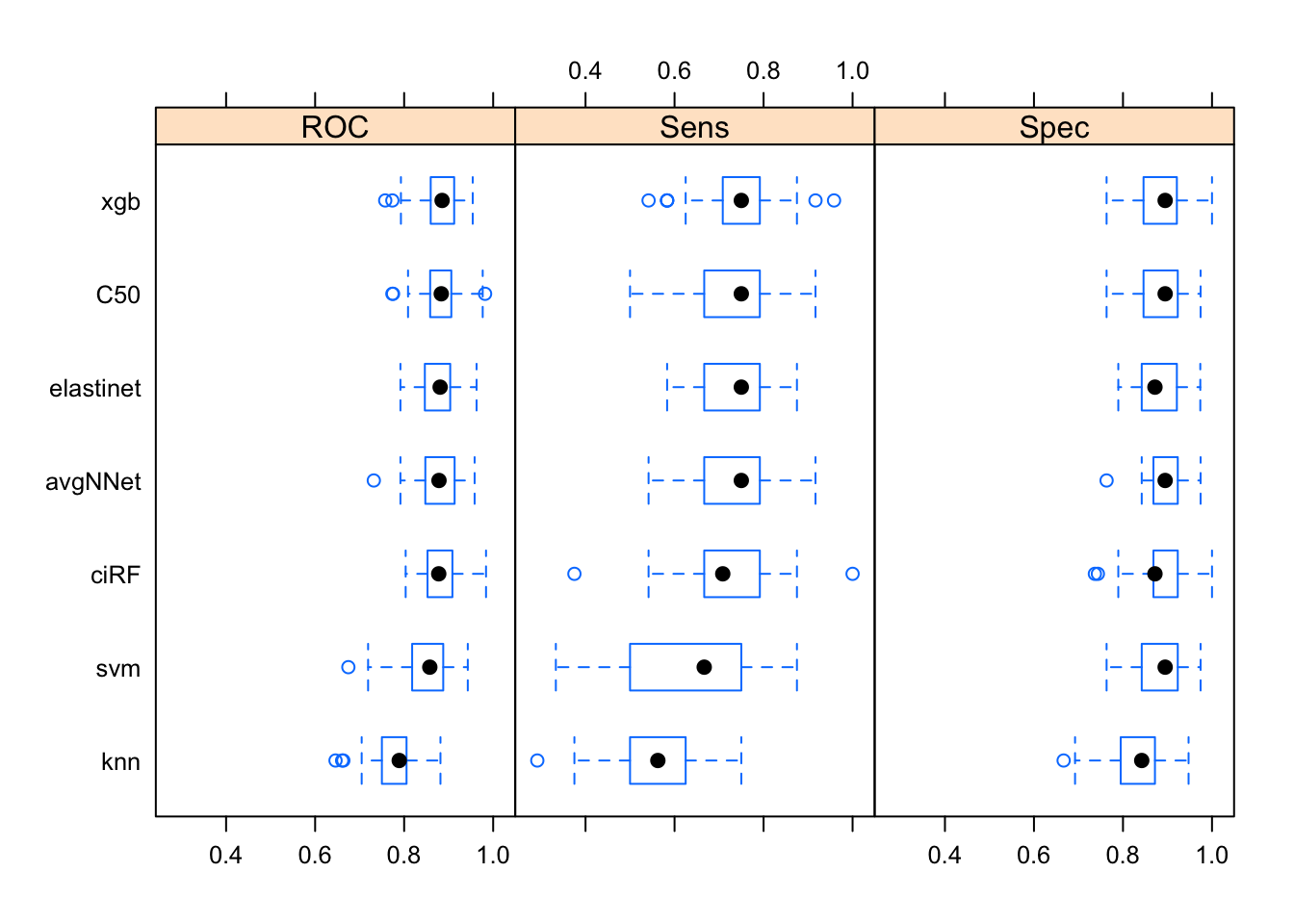One challenge in the plots above is drawing inferences from the plots above. Too many of the points above are really close to each other. It’s hard to tell if one model is any better than another model.

This is where `diff()` is a useful function. Basically, for each metric, all pair-wise differences are evaluated. The plot makes this quite intuitive. If the confidence level on the differences includes zero, the models are equal to each other. Similar to assessing a null hypothesis of (beta = 0) in a linear regression analysis.

Now, we can clearly see that if xgb is compared to it’s next 3 rivals, there isn’t really a statistically significant difference between the AUC ROC performance of that model against the others based on the cross-validations alone. No reason to pick over the others.

``````difValues <- diff(re)
dotplot(difValues)``````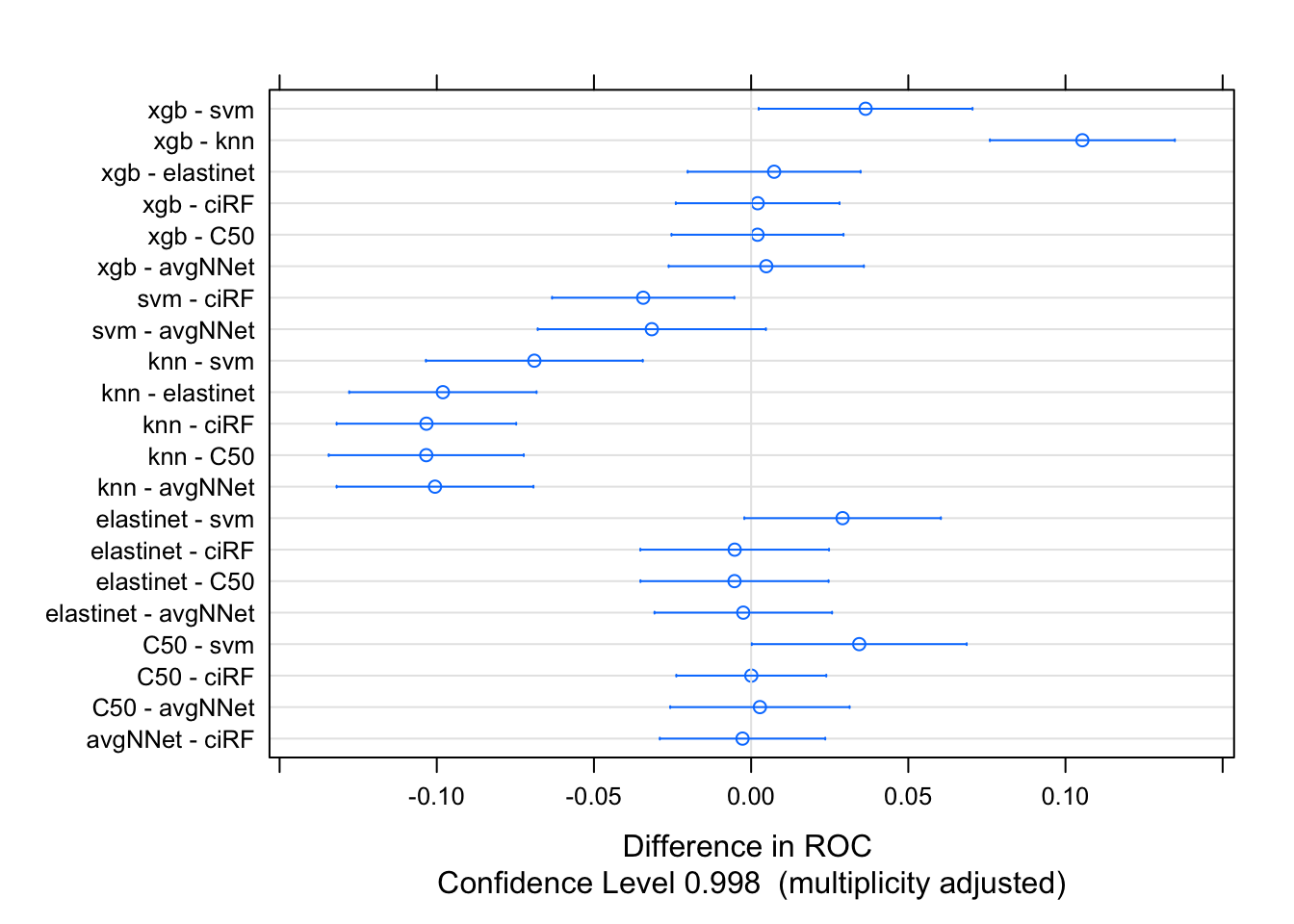# Test Set Evaluation

Of course, any data scientist worth his/her salt knows better than to use training set performances to fully evaluate and select the final model.

So, first re-trace all the steps and prepare the test dataset.

## Create test set

``````test.imp <- test.raw

#Embarked
test.imp\$Embarked[is.na(test.imp\$Embarked)]='S'

#Title
test.raw\$title <- str_extract(pattern = '[a-zA-Z]+(?=\\.)',string = test.raw\$Name)
test.imp\$title <- as.character(test.raw\$title)
test.imp\$title[test.imp\$title %in% c('Capt','Col','Major')] <- 'Officer'
test.imp\$title[test.imp\$title %in% c('Don','Dr','Rev','Sir','Jonkheer','Countess','Lady','Dona')] <- 'Royalty'
test.imp\$title[test.imp\$title %in% c('Mrs','Mme')] <- 'Mrs'
test.imp\$title[test.imp\$title %in% c('Ms','Mlle')] <- 'Miss'
test.imp\$title <- factor(test.imp\$title,levels = levels(train.imp\$title))

#Missing age
missing.age <- test.imp %>% filter(is.na(Age)) %>% dplyr::select(-Cabin,-Ticket,-Name)
age.predicted <- predict(rpartFit_ageimputation, newdata = missing.age)
test.imp\$Age[is.na(test.imp\$Age)] <- age.predicted

#Child
test.imp\$child <- 0
test.imp\$child[test.imp\$Age<18] <- 1

#Young/old
test.imp\$Seniors <- ifelse(test.imp\$Age>60,1,0)

#Family Related
test.imp\$TotalFam <- test.imp\$SibSp + test.imp\$Parch + 1
test.imp\$LargeFamily <- ifelse(test.imp\$TotalFam>4,1,0)
test.imp\$Name <- NULL

#Cabin & Deck
test.imp\$CabinMissing <- as.numeric(is.na(test.raw\$Cabin))
test.imp\$CabinCode <- map_chr(test.raw\$Cabin,~str_split(string = .x,pattern = '')[])
test.imp\$CabinCode[is.na(test.imp\$CabinCode)] <- 'U'
test.imp\$CabinCode <- factor(test.imp\$CabinCode,levels = levels(train.imp\$CabinCode))
test.imp\$CabinNum <- as.numeric(map_chr(test.raw\$Cabin,~str_split(string = .x,pattern = '[a-zA-Z]')[]))
test.imp\$CabinNum <- map_int(test.imp\$CabinNum, ~as.integer(str_split(.x,pattern = '',simplify = T)))
test.imp\$CabinNum[is.na(test.imp\$CabinNum)] <- 0

test.imp\$TopDeck <- ifelse(test.imp\$CabinCode %in% c('A','B'),1,0)
test.imp\$MidDeck <- ifelse(test.imp\$CabinCode %in% c('C','D'),1,0)
test.imp\$LowerDeck <- ifelse(test.imp\$TopDeck==0 & test.imp\$MidDeck==0 ,1,0)

test.imp\$NumberofCabins <- map_int(test.raw\$Cabin,~str_split(string = .x,pattern = ' ')[] %>% length)
test.imp\$Cabin <- NULL

# Ticket
test.imp %<>%
mutate(
Ticket = str_to_upper(Ticket) %>%
str_replace_all(pattern = regex(pattern = '[.\\/]'),replacement = ''),
TicketNum = str_extract(Ticket,pattern = regex('([0-9]){3,}')),
TicketNumStart = map_int(TicketNum,~as.integer(str_split(.x,pattern = '',simplify = T))),
TicketNumLen = map_int(TicketNum,~dim(str_split(.x,pattern = '',simplify = T))),
TicketChar = str_extract(Ticket,pattern = regex('^[a-zA-Z/\\.]+'))
) %>%
mutate(
TicketChar = map_chr(.x=TicketChar,
.f=~str_split(string=.x, pattern = '',simplify = T))
) %>%
mutate(
TicketChar = ifelse(is.na(TicketChar),'U',TicketChar),
TicketNumStart = ifelse(is.na(TicketNumStart),0,TicketNumStart),
TicketNumLen = ifelse(is.na(TicketNumLen),0,TicketNumLen),
) %>%
mutate(
TicketChar = as.factor(TicketChar),
TicketNumStart = factor(TicketNumStart,levels = seq(0,9,1)),
TicketNumLen = as.factor(TicketNumLen)
)
test.imp\$Ticket <- NULL
test.imp\$TicketNum <- NULL``````

## Predict test results

Now, predict the results using all the modelFits created in the previous section.

``````elastinetPred   <- predict(object = glmnetFit, newdata = test.imp)
xgbPred         <- predict(object = xgbFit,    newdata = test.imp)
c5Pred          <- predict(object = c5Fit,     newdata = test.imp)
knnPred         <- predict(object = knnFit,    newdata = test.imp)
svmPred         <- predict(object = svmFit,    newdata = test.imp)
avNNPred        <- predict(object = avNnetFit, newdata = test.imp)
ciRFPred        <- predict(object = ciRFFit,   newdata = test.imp)``````

Predictions are done; now let’s investigate the results. Extracting confusion matrices is made simple using `caret`. Comparing all the results is made easy if we leverage the tools in `purrr`.

``````xtab <- table(xgbPred,test.imp\$Survived)
xgbCM <- caret::confusionMatrix(xtab)

xtab <- table(elastinetPred,test.imp\$Survived)
elastinetCM <- caret::confusionMatrix(xtab)

xtab <- table(c5Pred,test.imp\$Survived)
c5CM <- caret::confusionMatrix(xtab)

xtab <- table(knnPred,test.imp\$Survived)
knnCM <-caret::confusionMatrix(xtab)

xtab <- table(svmPred,test.imp\$Survived)
svmCM <-caret::confusionMatrix(xtab)

xtab <- table(avNNPred,test.imp\$Survived)
avNNCM <-caret::confusionMatrix(xtab)

xtab <- table(ciRFPred,test.imp\$Survived)
ciRFCM <-caret::confusionMatrix(xtab)

CM_list <- list(xgbCM, elastinetCM, c5CM, knnCM, svmCM, avNNCM, ciRFCM)

compiled_results <- tibble(
models = c('xgb','elastinet','C5.0','knn','svm', 'avgNN', 'ciRF'),
accuracy = map_dbl(CM_list,~.x\$overall),
kappa = map_dbl(CM_list,~.x\$overall),
sensitivity = map_dbl(CM_list,~.x\$byClass),
specificity = map_dbl(CM_list,~.x\$byClass),
F1 = map_dbl(CM_list,~.x\$byClass)
)
compiled_results %>% arrange(accuracy,kappa)``````
``````## # A tibble: 7 x 6
##   models    accuracy kappa sensitivity specificity    F1
##   <chr>        <dbl> <dbl>       <dbl>       <dbl> <dbl>
## 1 knn          0.737 0.413       0.520       0.872 0.602
## 2 C5.0         0.808 0.581       0.667       0.896 0.727
## 3 ciRF         0.808 0.585       0.686       0.884 0.733
## 4 xgb          0.816 0.598       0.676       0.902 0.738
## 5 avgNN        0.823 0.616       0.696       0.902 0.751
## 6 svm          0.846 0.665       0.725       0.921 0.783
## 7 elastinet    0.850 0.675       0.745       0.915 0.792``````

Of course, the easiest way to look at the results is visually. Elastinet and svm are the clear winners here, with 84%+ accuracy. The kappa and F1 scores together also show them are clear winners. This is what’s interesting about using the test datasets. The cross-validation training results had xgb, C50, ciRF, avgNNet and elastinet all almost equally as good. However, the test results show a very clear winner indeed.

``````dotplot(reorder(models,accuracy)~accuracy,compiled_results, main = 'Accuracy (Test Set Performance)')
ggplot(compiled_results, aes(F1, accuracy)) +
geom_point(color = 'blue',shape=1) +
geom_text_repel(aes(label = models),
max.iter=1e2,segment.size=.3,
force=1) +
theme_bw()+
labs(x='F1',y='kappa', title='Kappa vs F1 (Test Set Performance)')``````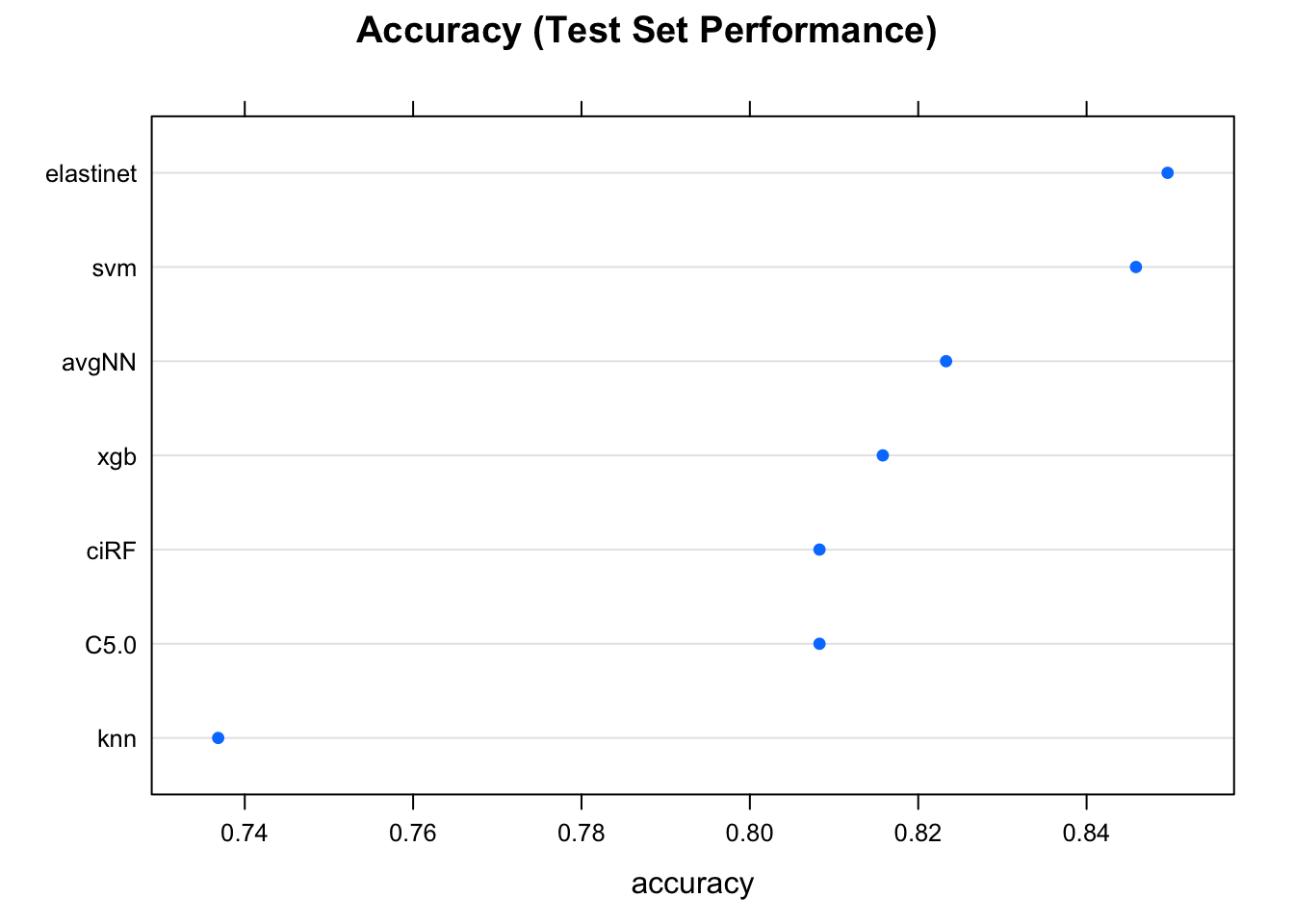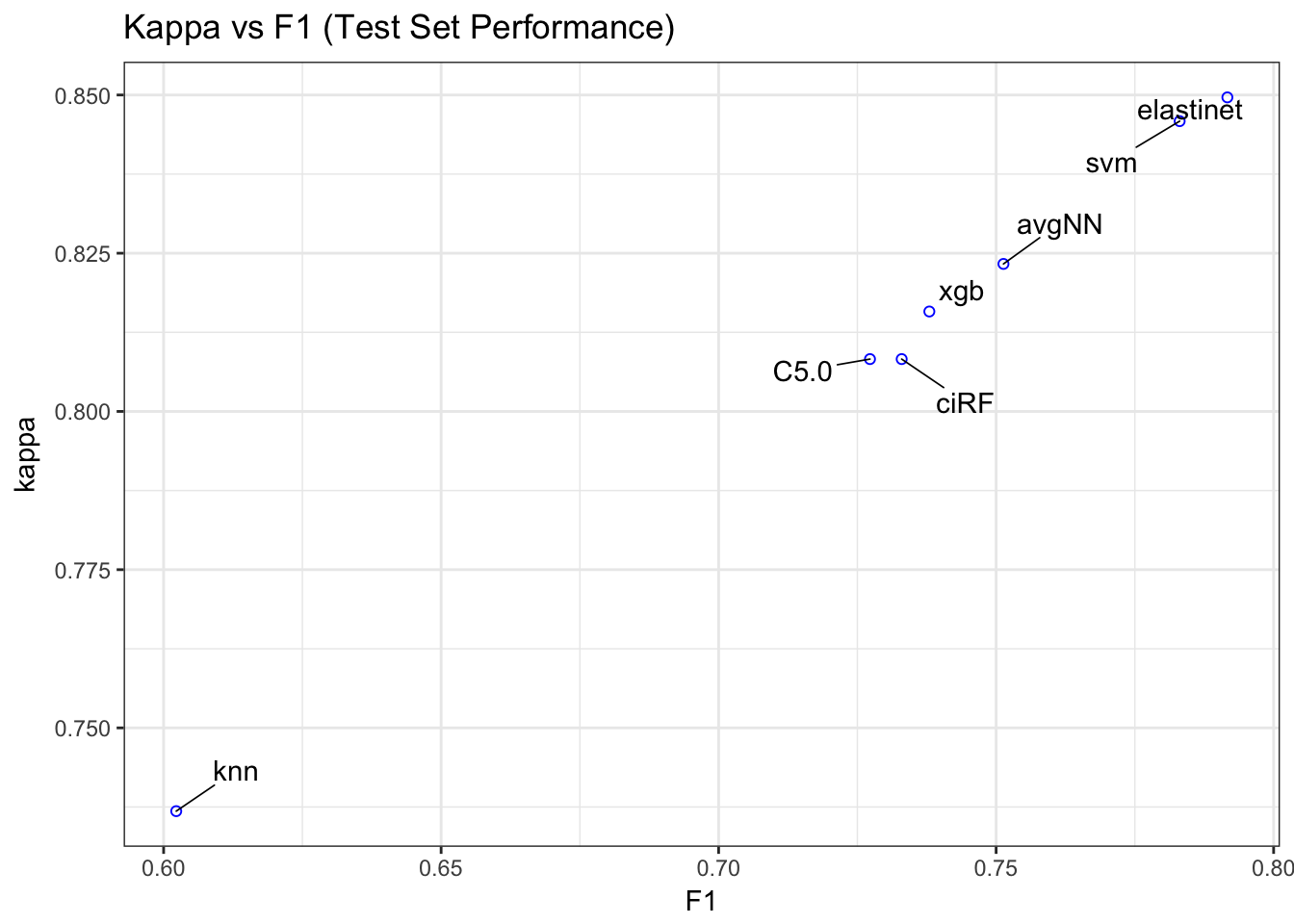# Kaggle Performance

The top few models take me to a 72% - 76% score on Kaggle. It isn’t the top model there of course, but not bad for a 1st Kaggle competiton attempt.

1. I think this approach depends on the academic background and the industry of the analyst. Prof Srinivasan, and my mentor at work both have strong statistical academic backgrounds, and both believe in thorough EDA of the data. I’ve also noticed this approach from individuals in the banking & insurance industry - perhaps due to regulatory requirements. On the other hand, folks trained in computer science and algorithmic data science tend to underplay the importance of thorough EDA.

2. To iterate variable names in ggplot, use `ggplot(...)+aes_string(...)` in place of `ggplot(...,aes(...))`.

3. Read more about beanplots here: https://cran.r-project.org/web/packages/beanplot/vignettes/beanplot.pdf